# ICSE Class 10 Maths Question Paper Solution 2018

Students who are aiming to score high marks in the Maths subject must solve the previous year question paper. Here we have provided the ICSE Class 10 Maths Question Paper 2018 along with the Solutions. Solving the ICSE class 10 previous year maths question papers will not only help them in understanding the paper pattern but also help them in evaluating their performance. By solving the ICSE Class 10 Maths Question Paper they will get to know where they are committing the mistake and then in such a way they can work on those points to improve their performance in the exam. The ICSE Class 10 Maths Question Paper 2018 Solutions contains the answers explained in steps along with the marking scheme for students’ easy understanding.

The ICSE Class 10 Maths 2018 exam was conducted on 27th February 2018. The exam started at 11am and students were allotted 2 hours 30 minutes of time duration to finish the paper. Students can download the ICSE Class 10 Maths Question Paper Solution 2018 PDF from the link below.

Students can have a look at the ICSE Class 10 Maths Question Paper Solution 2018 below: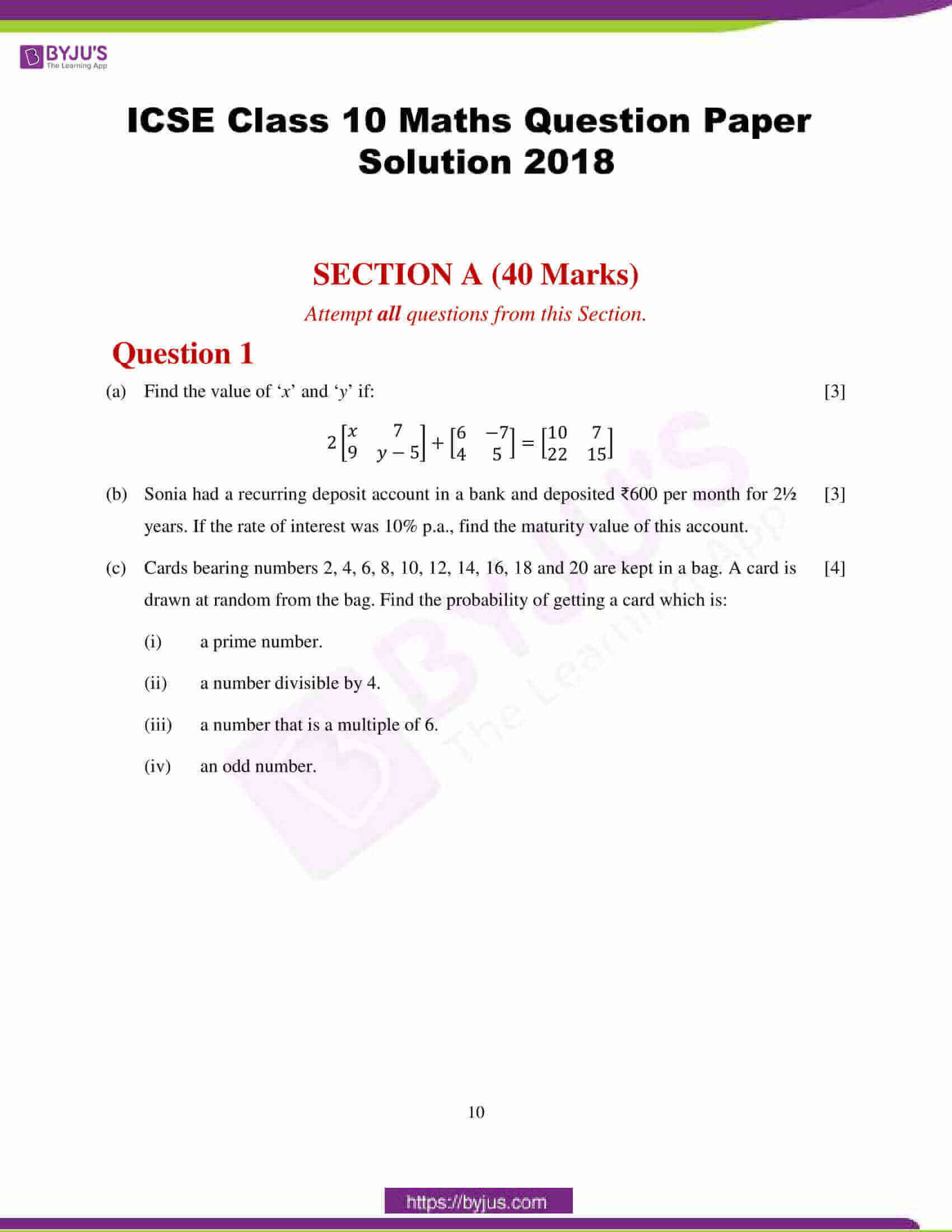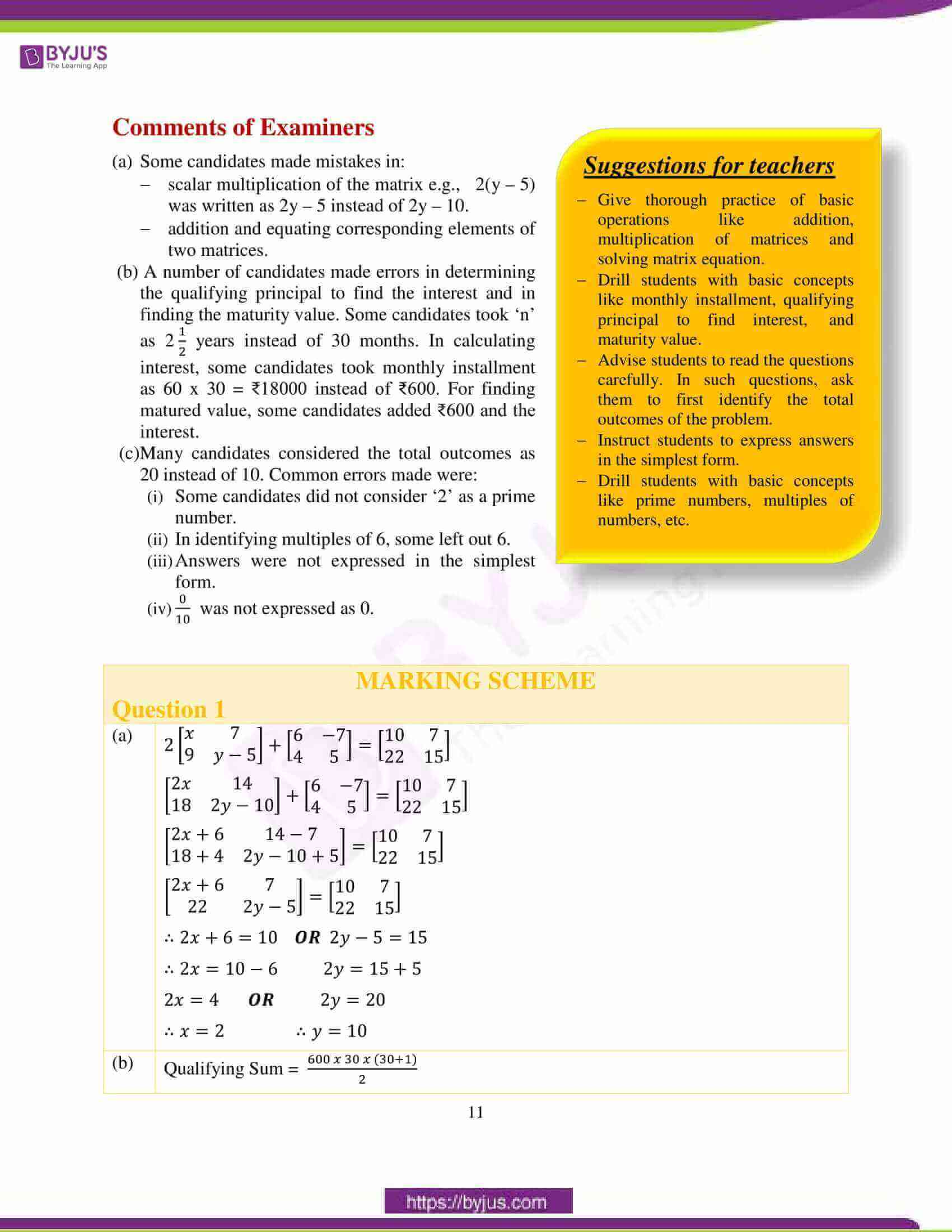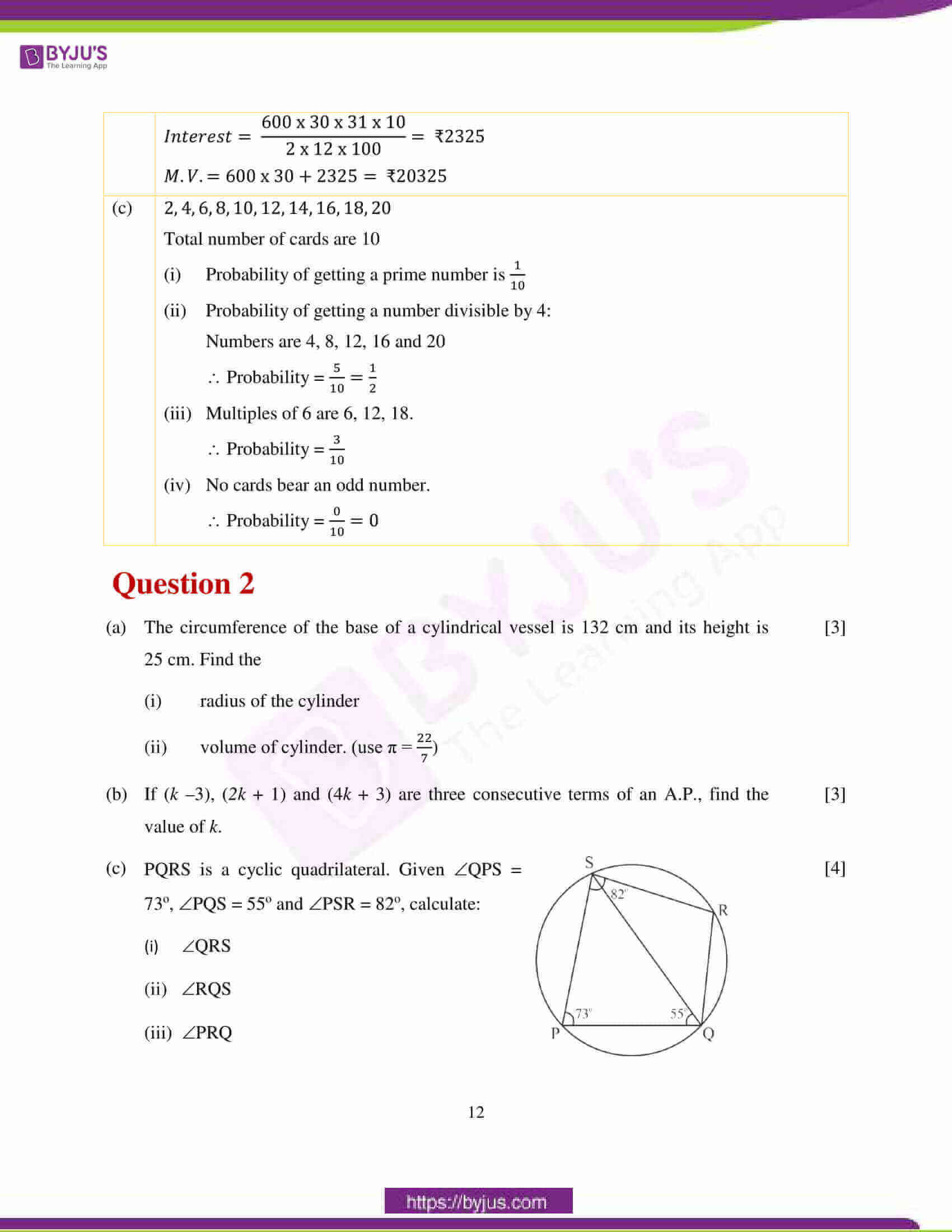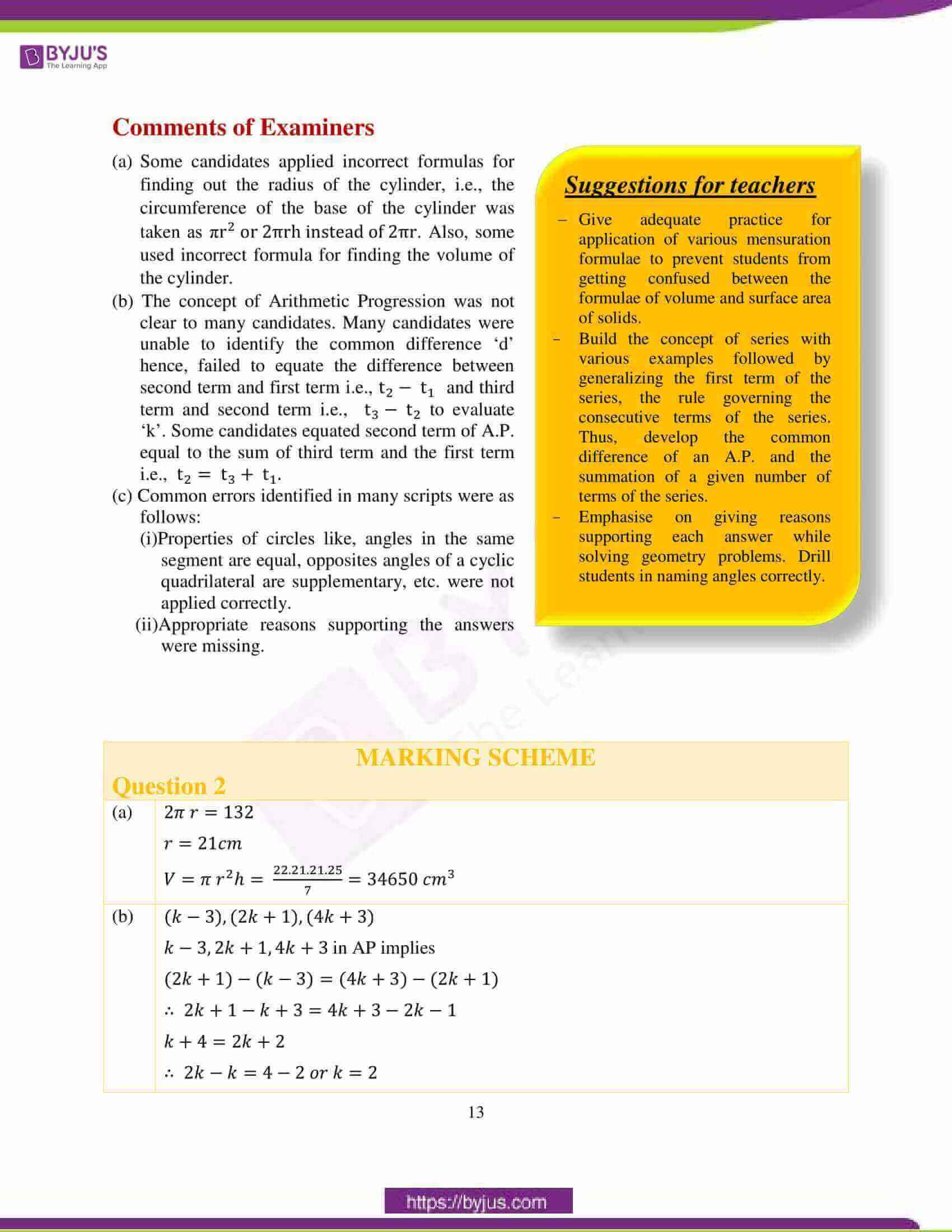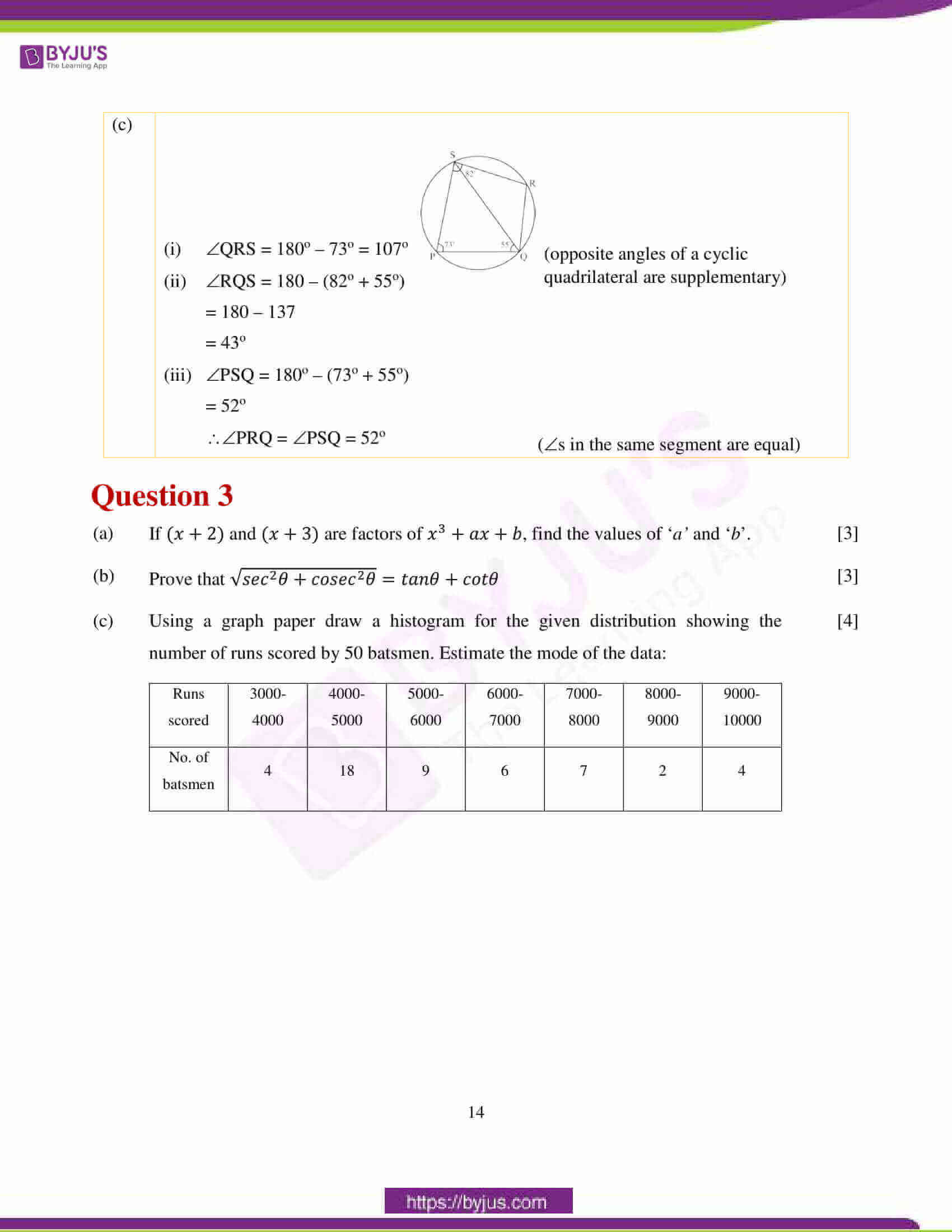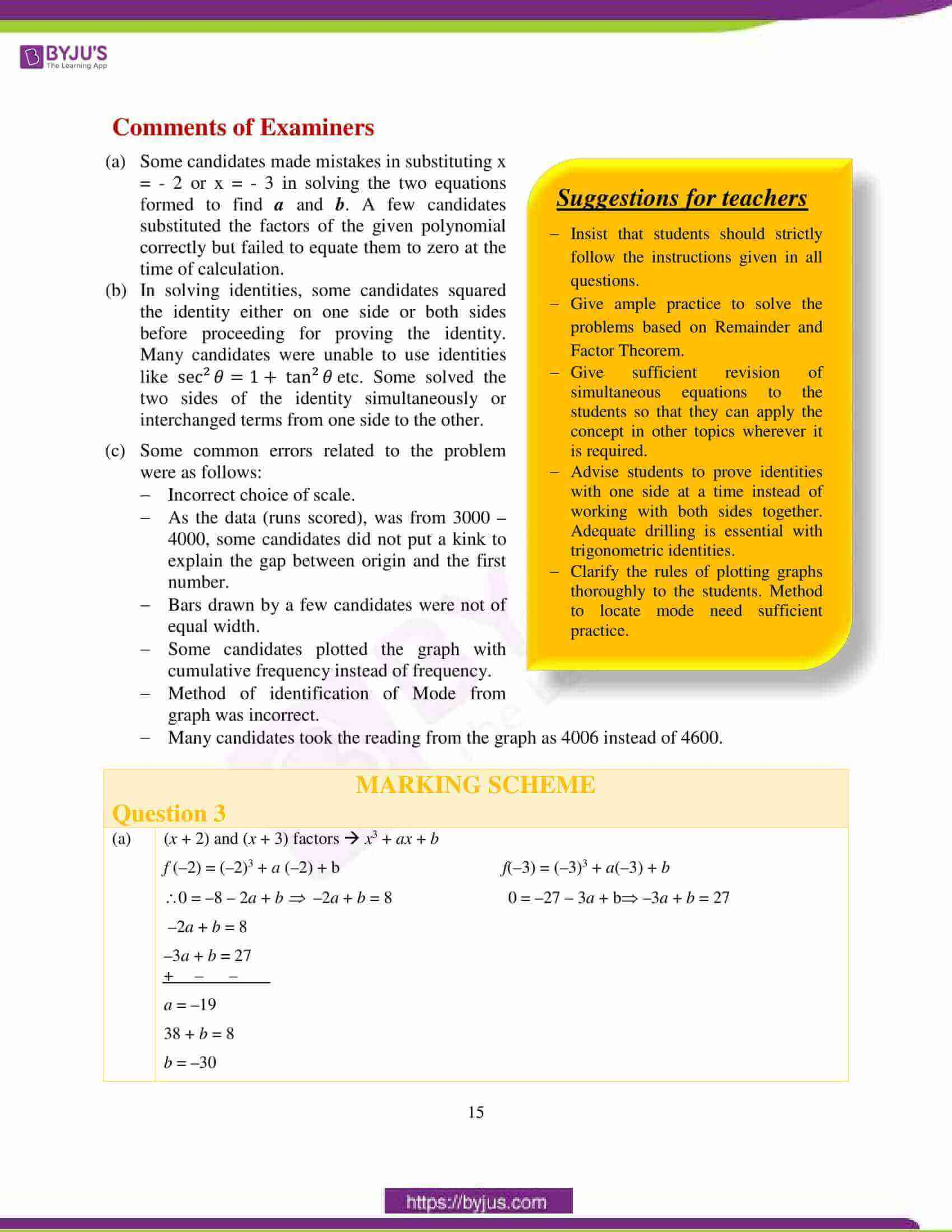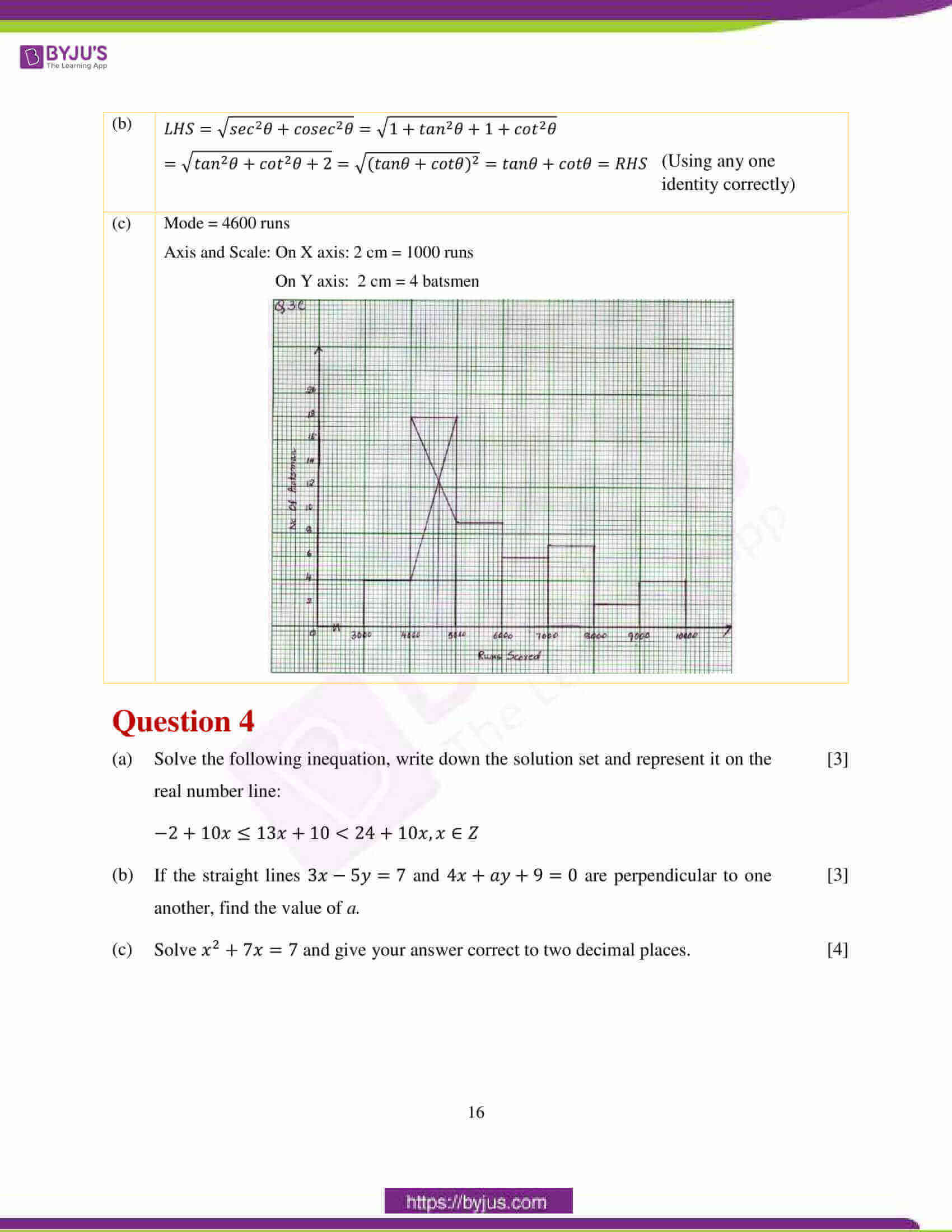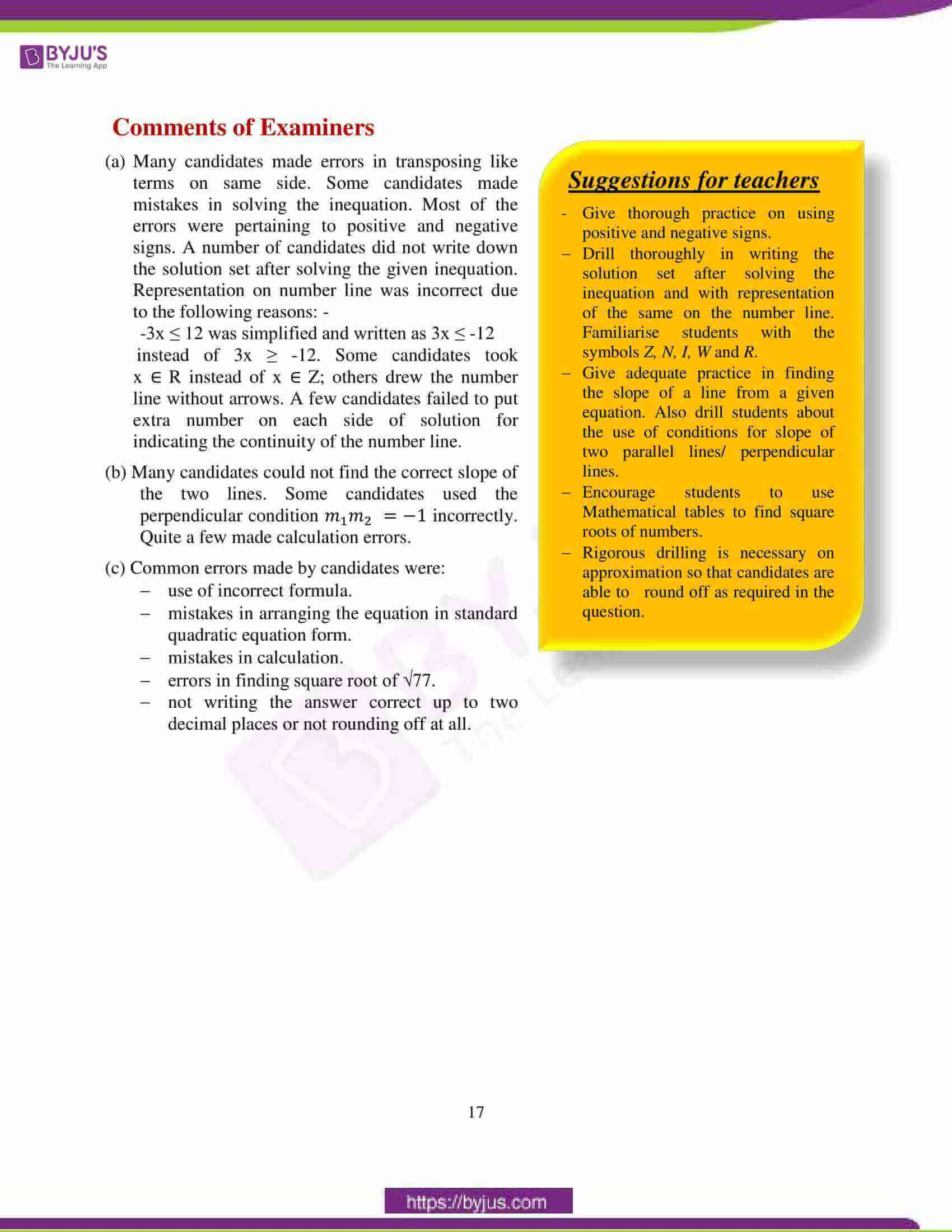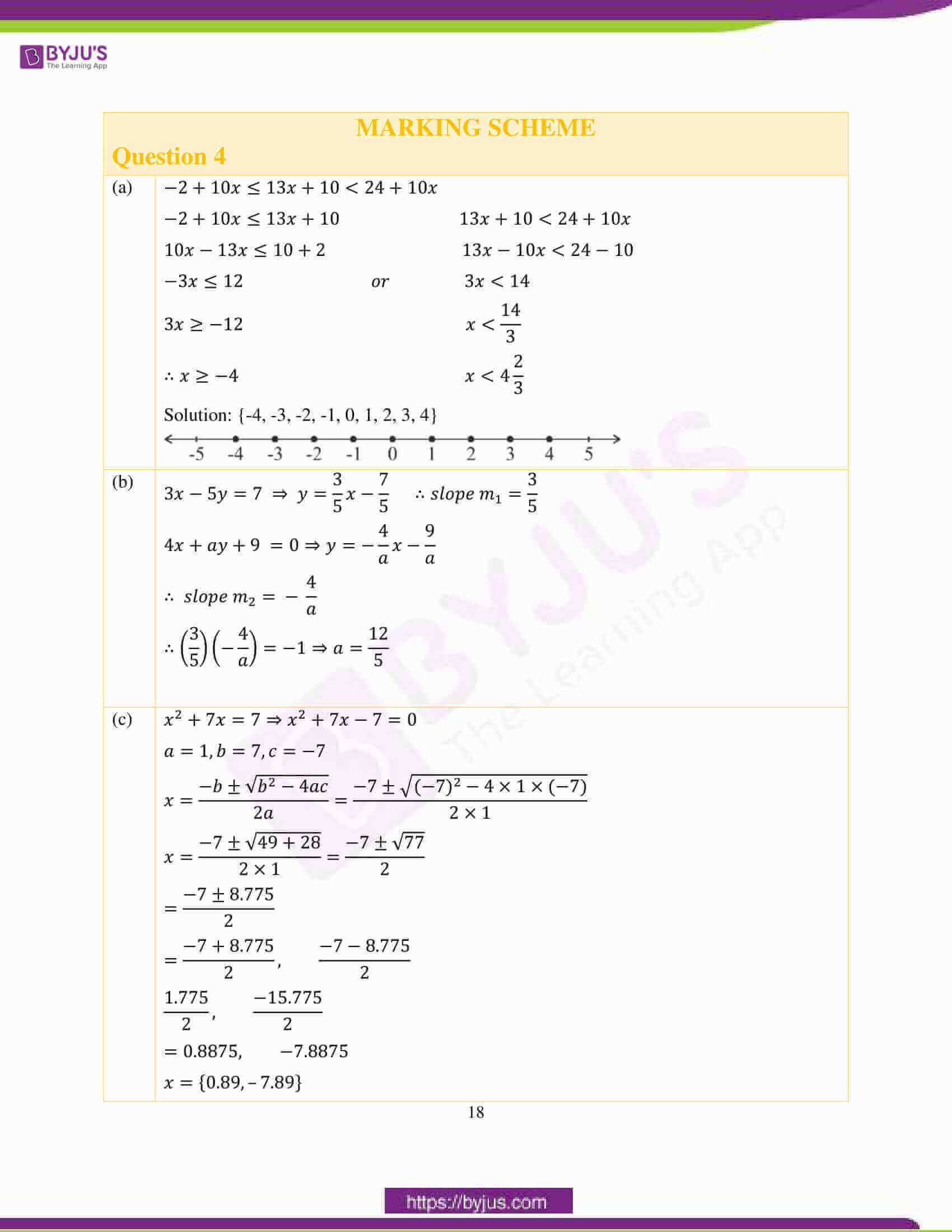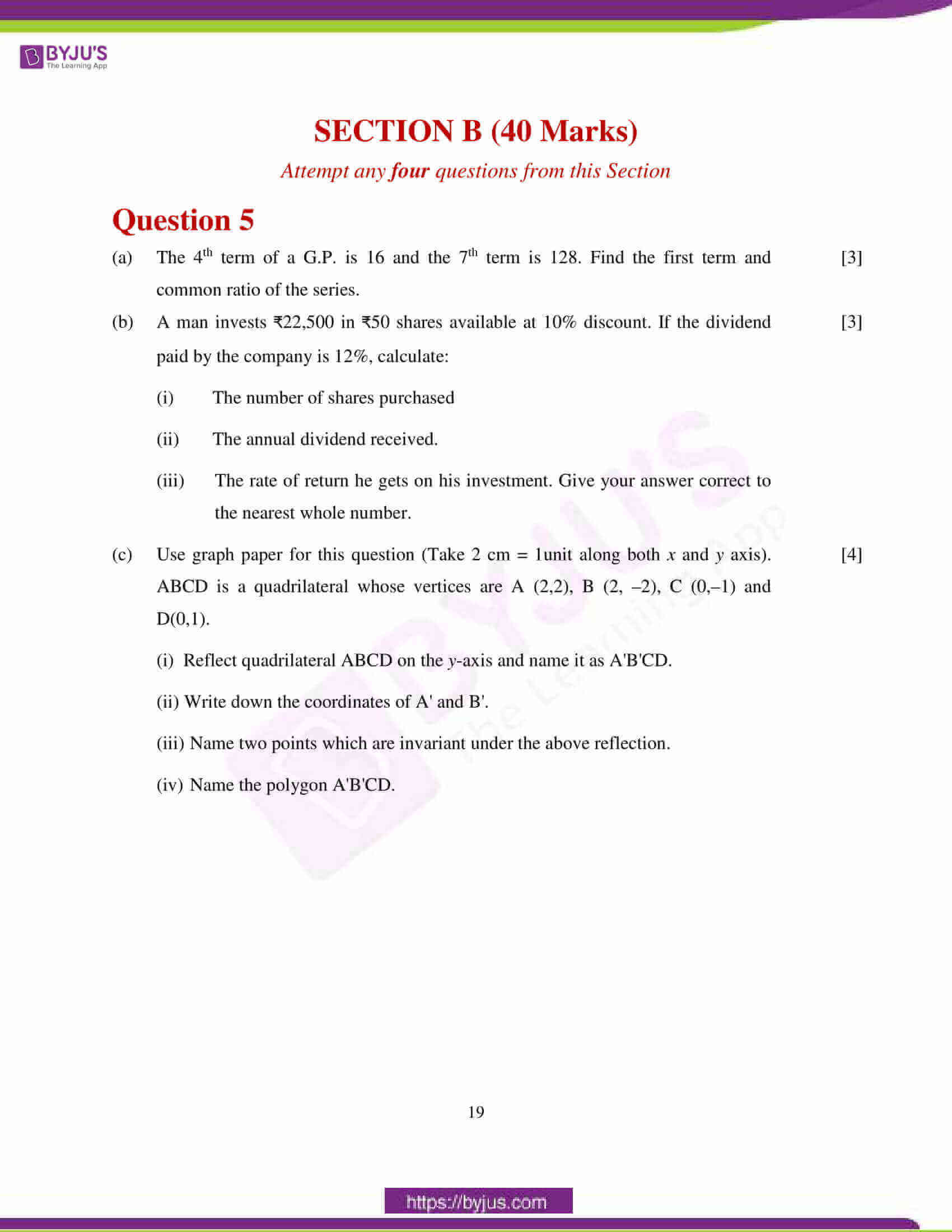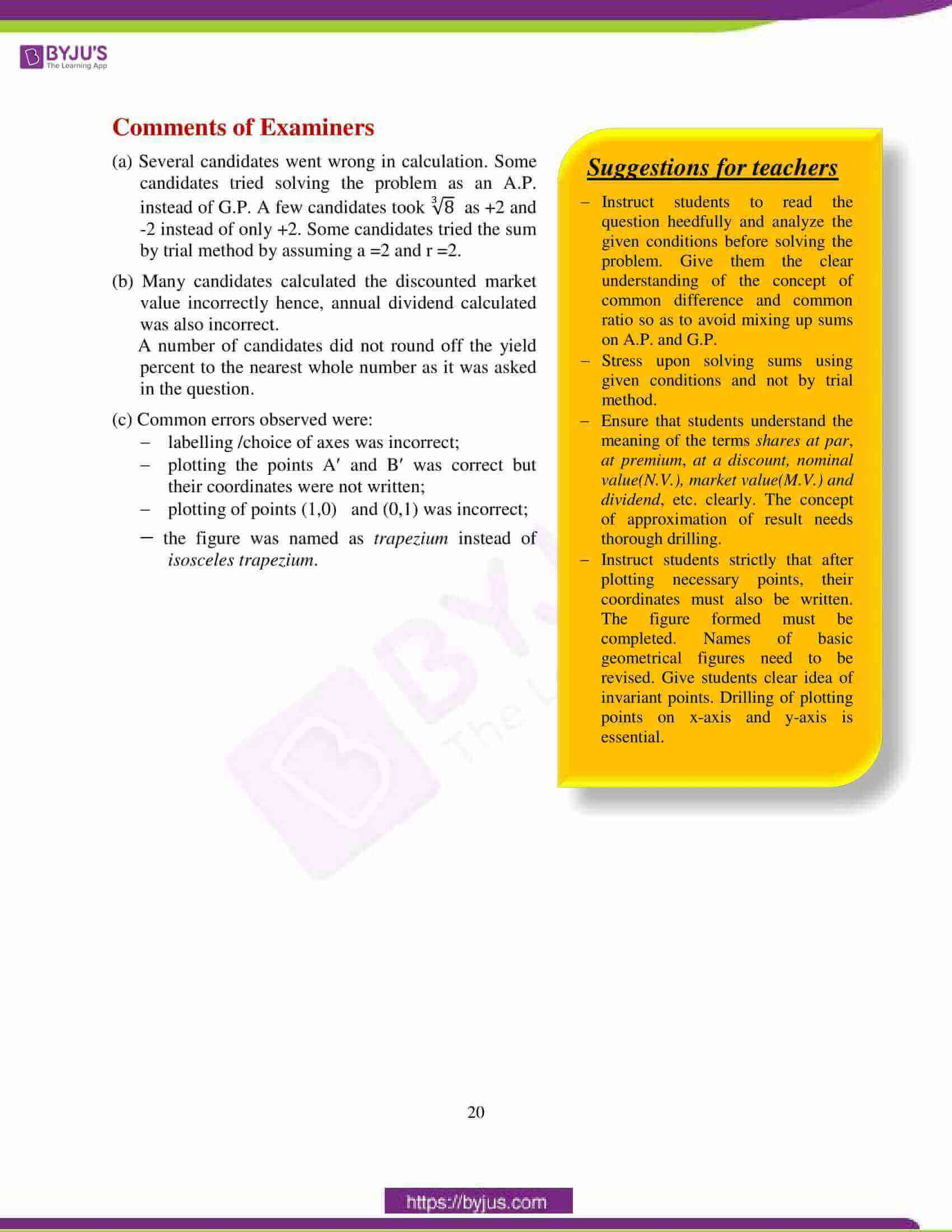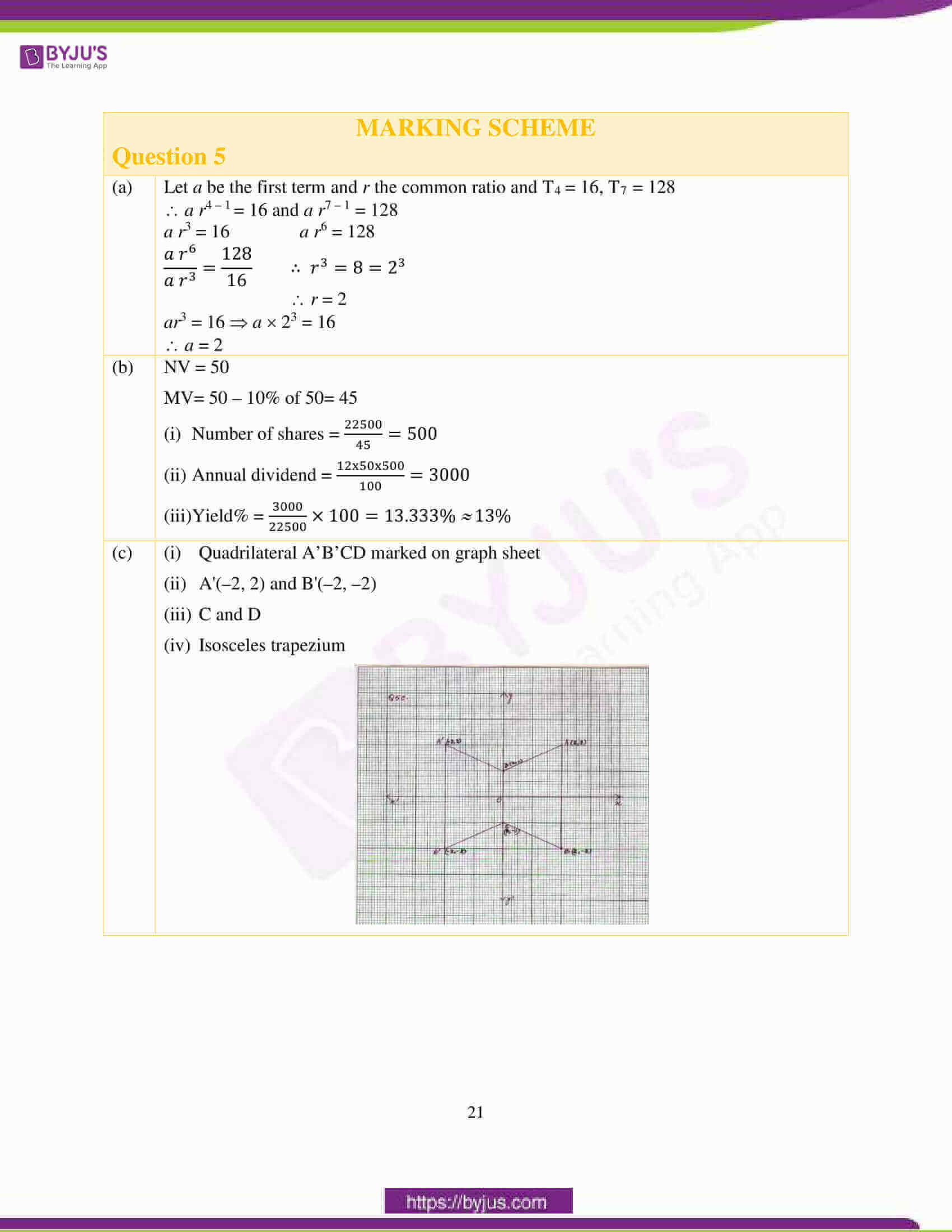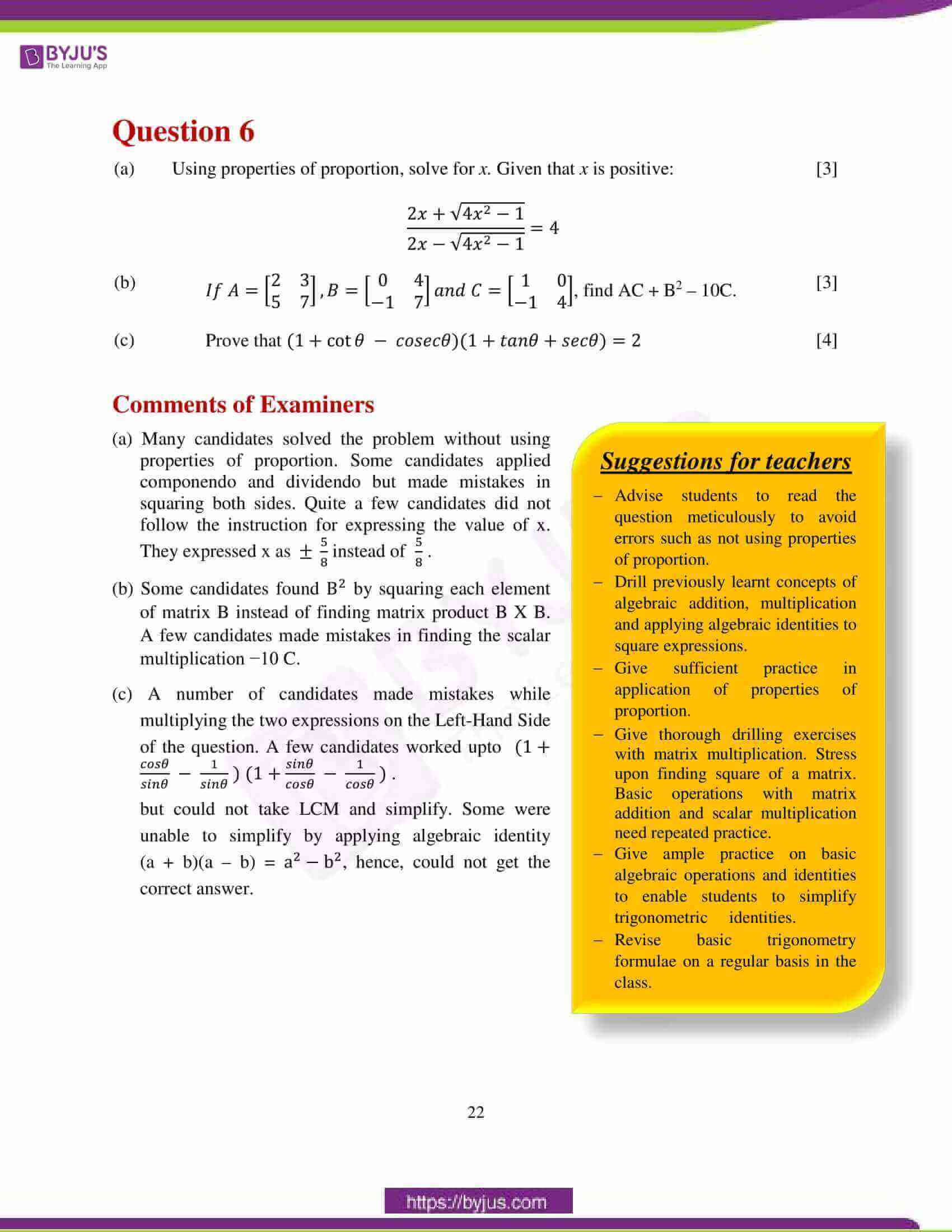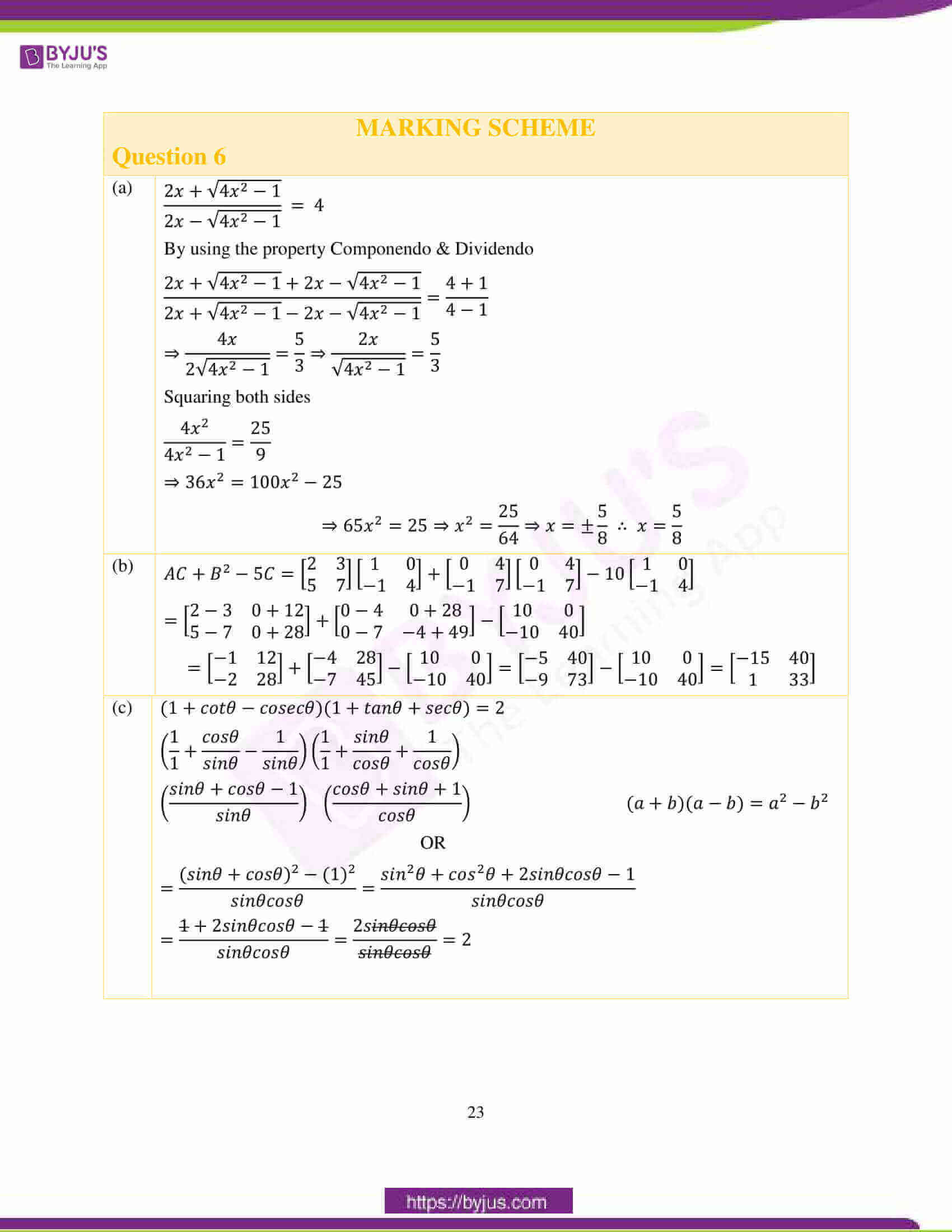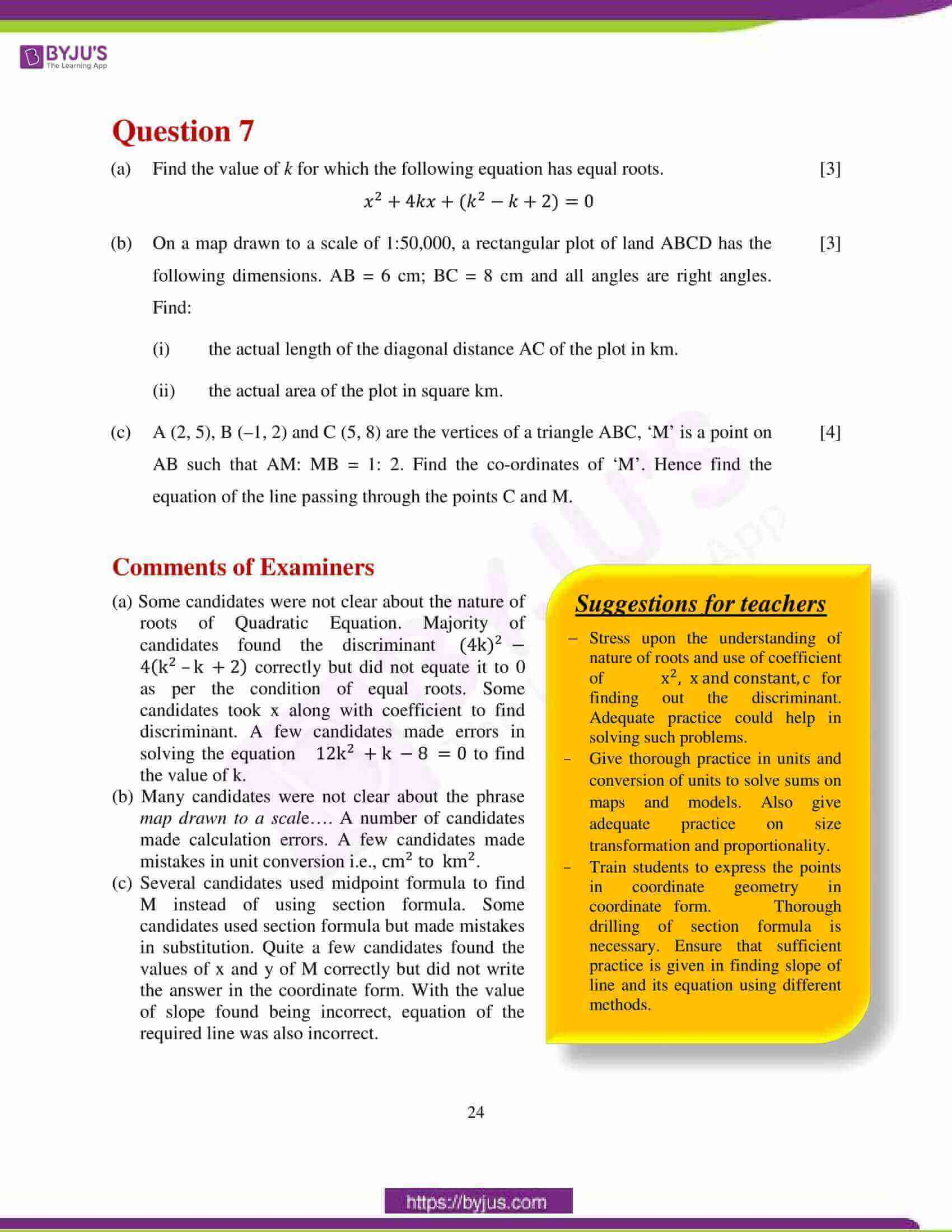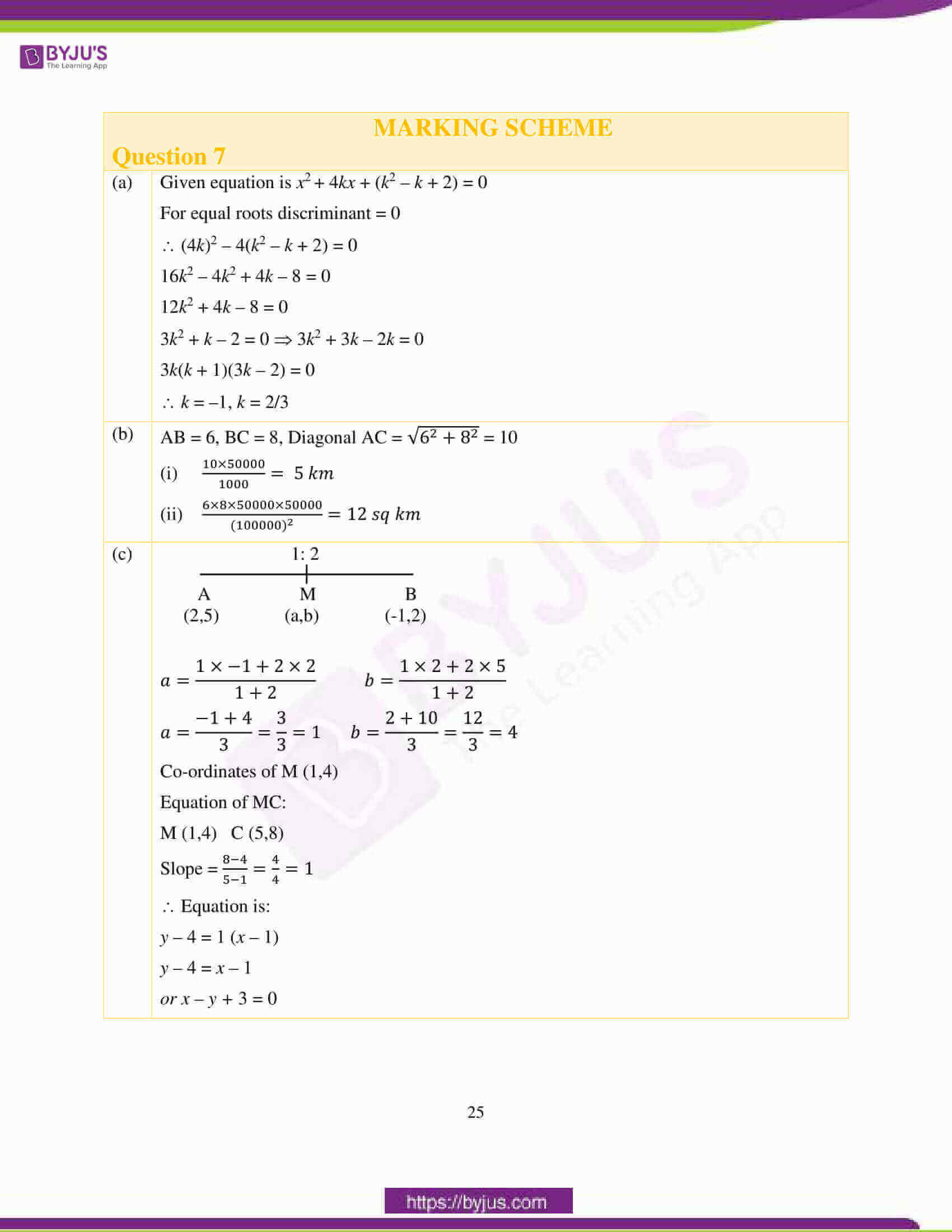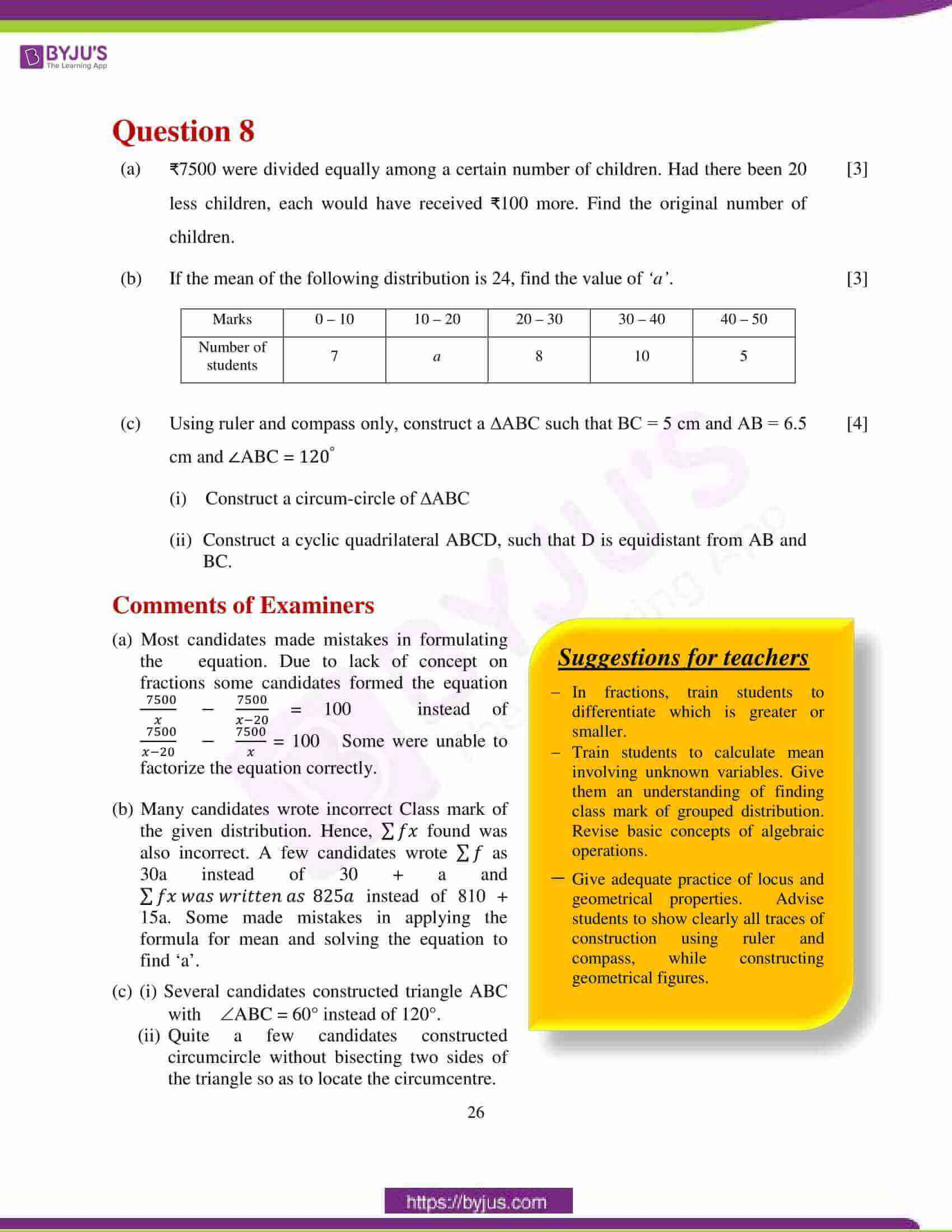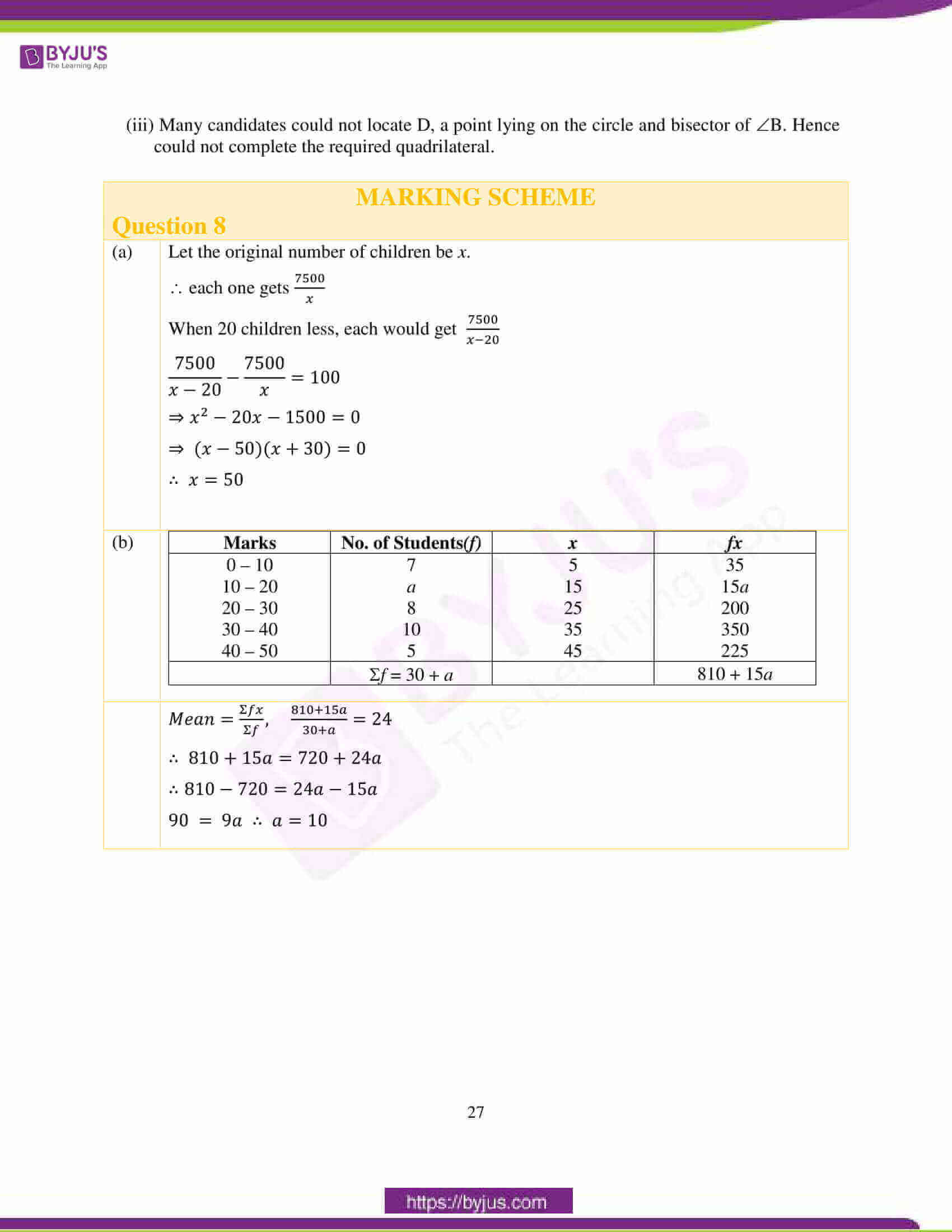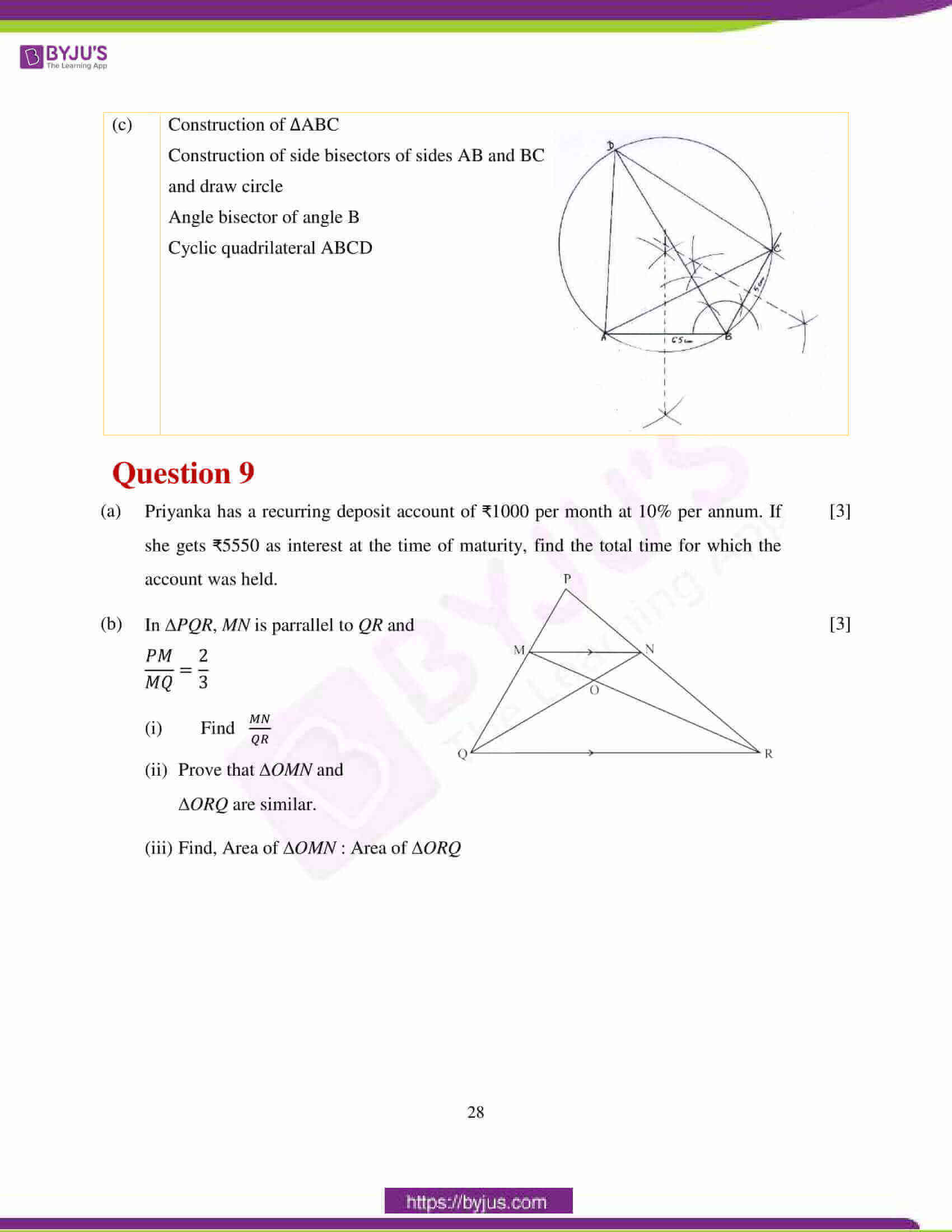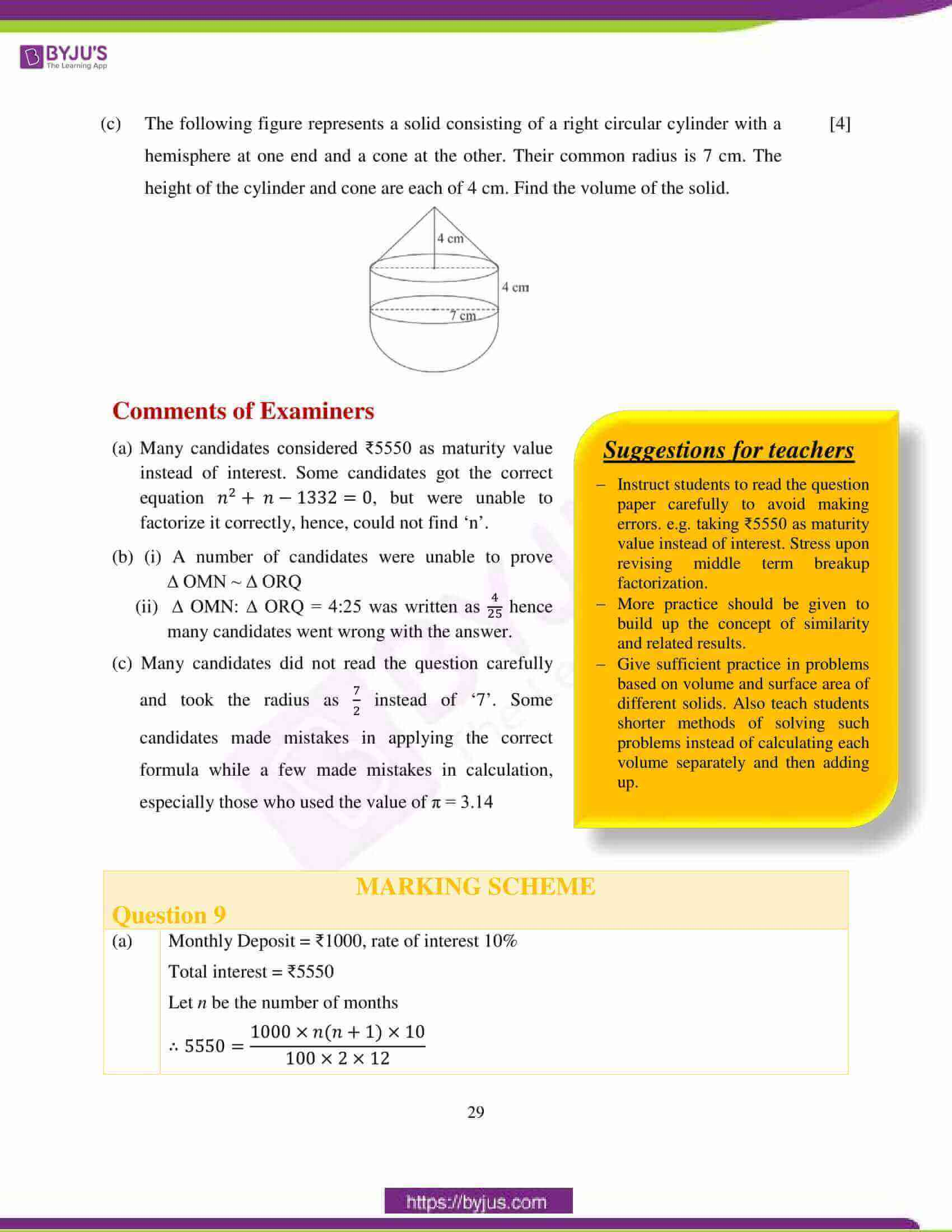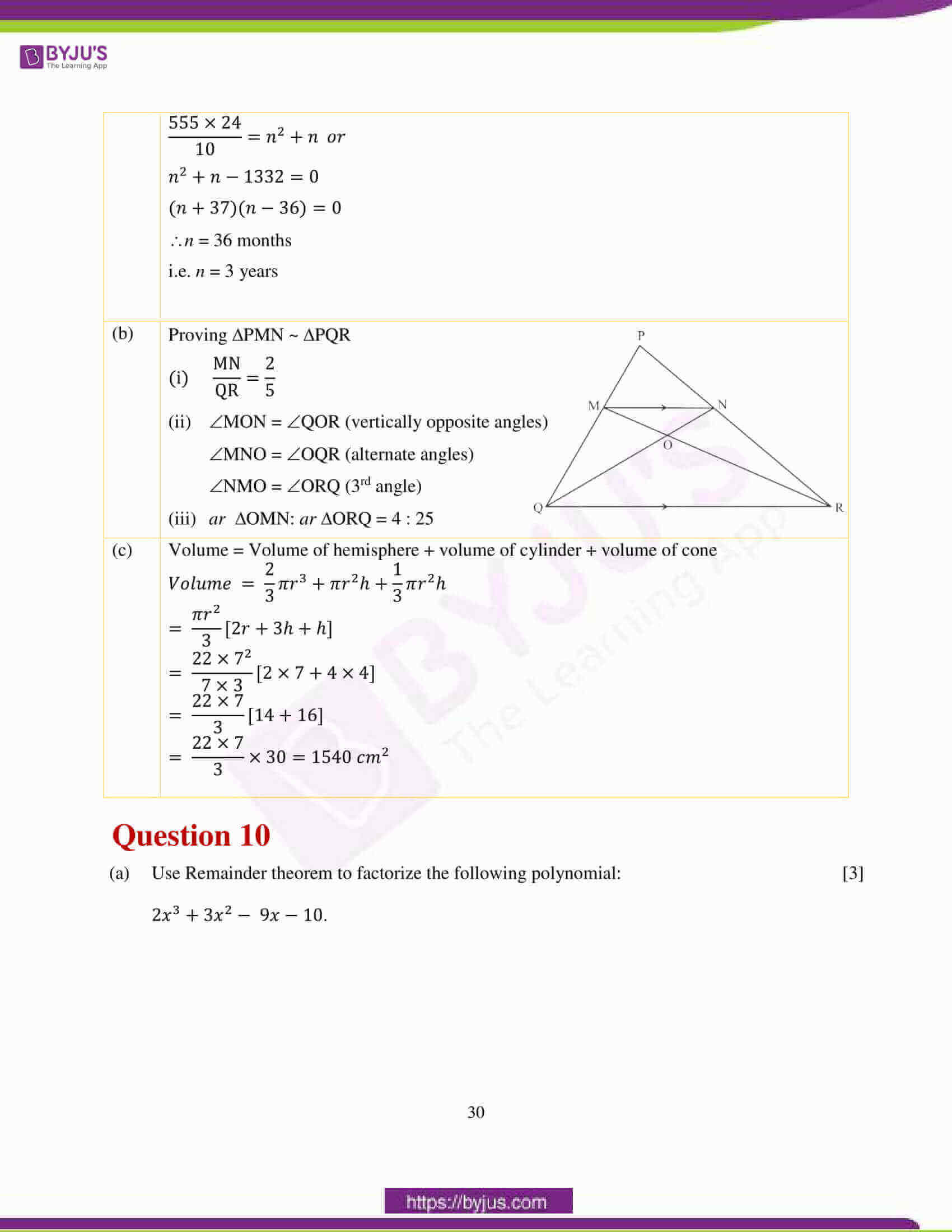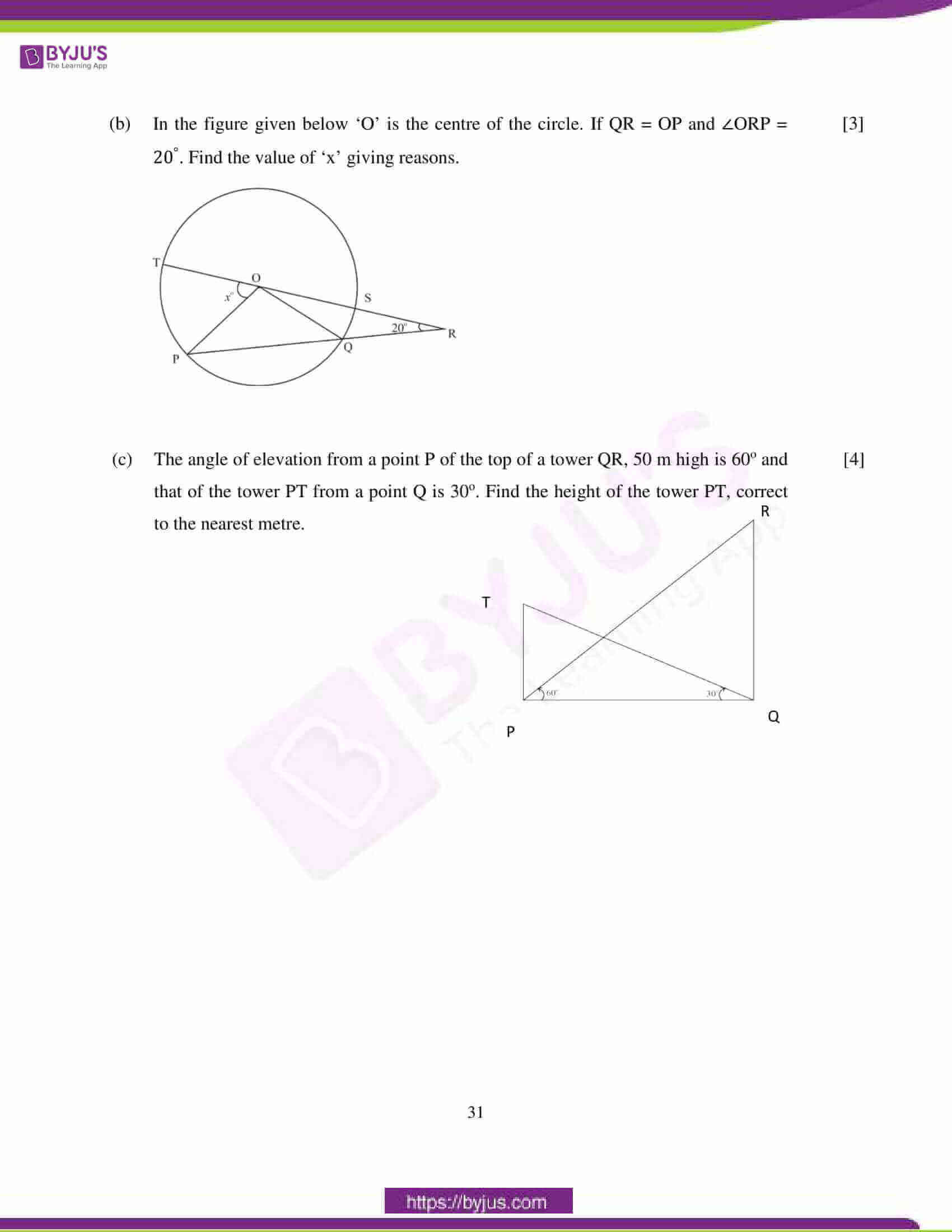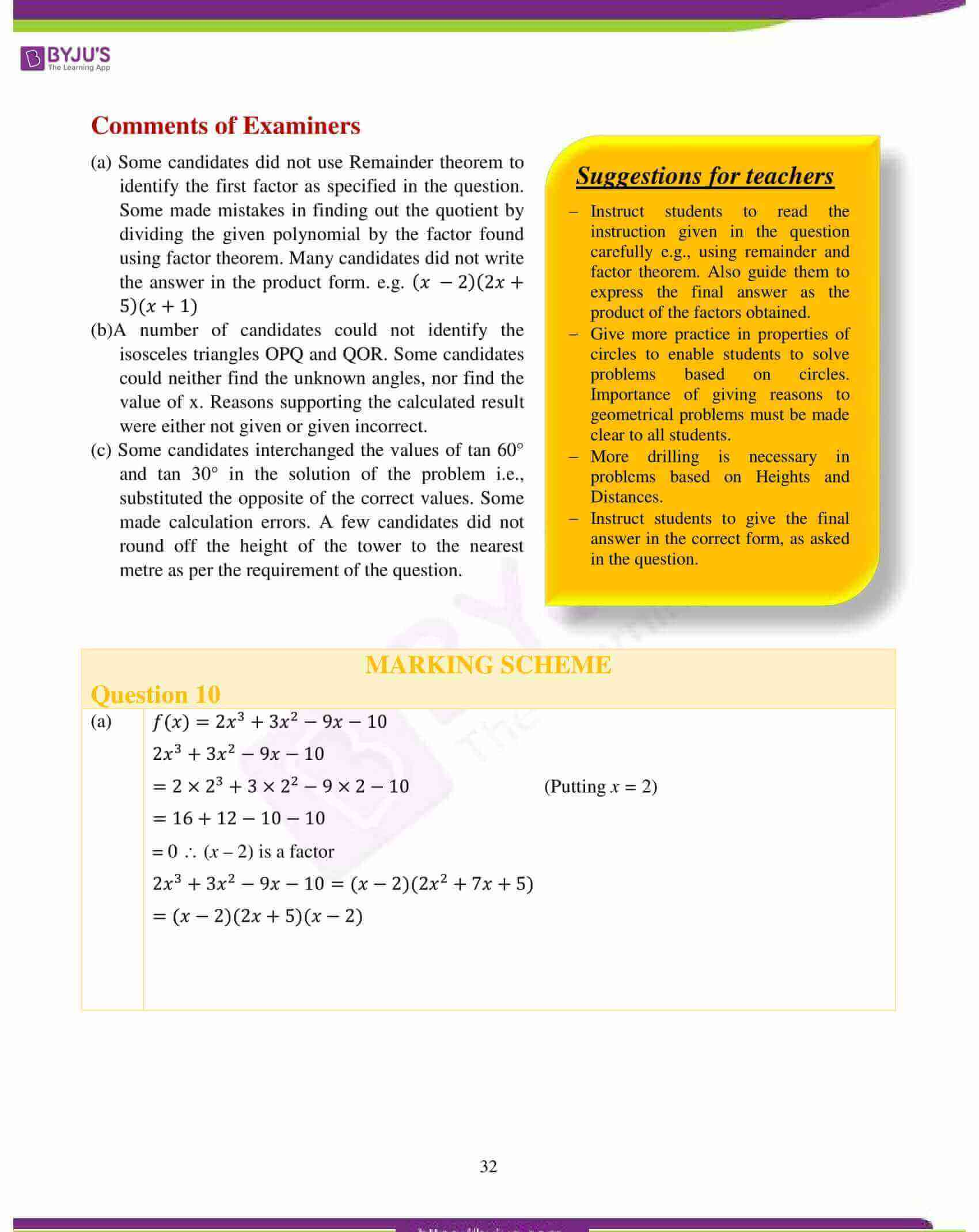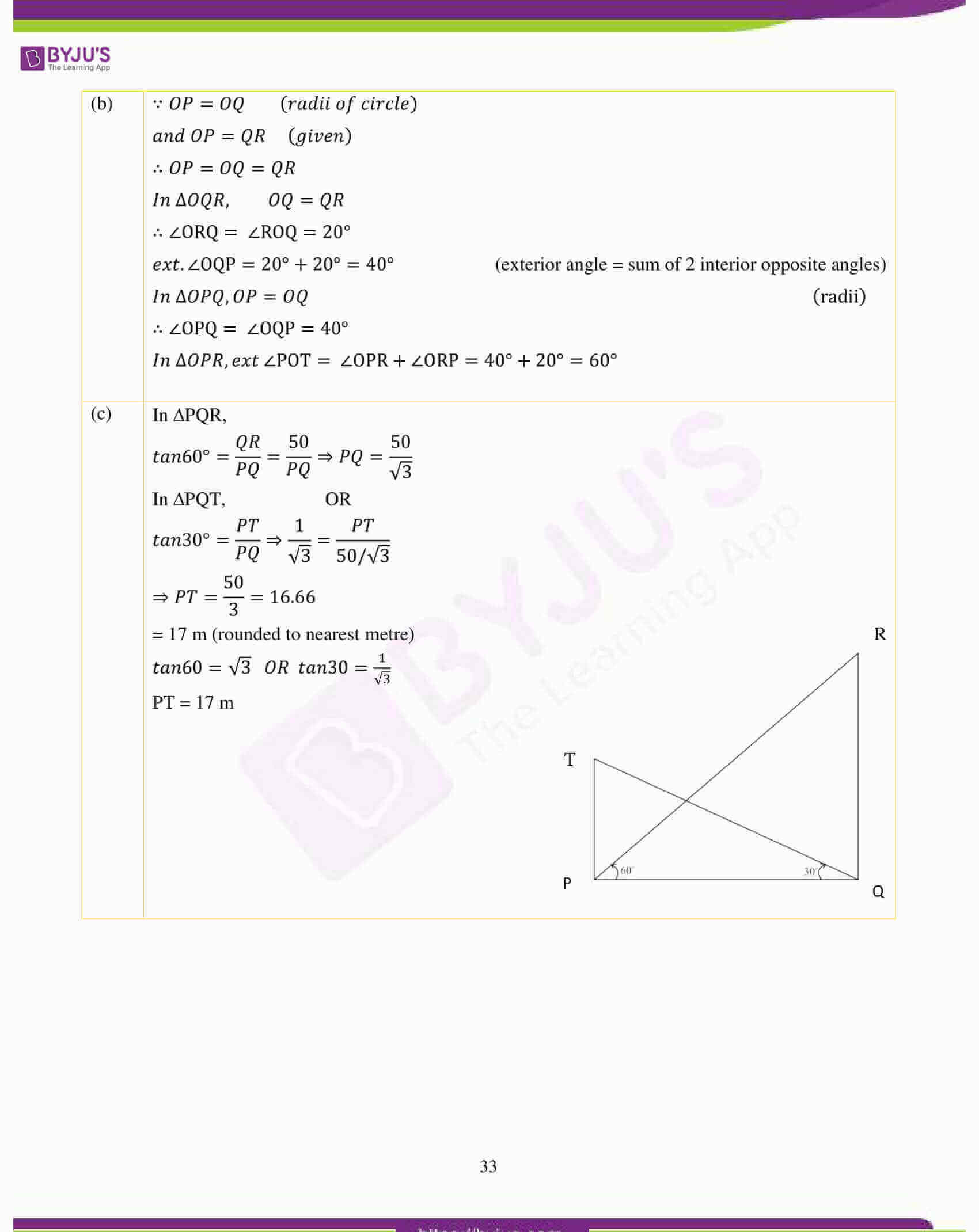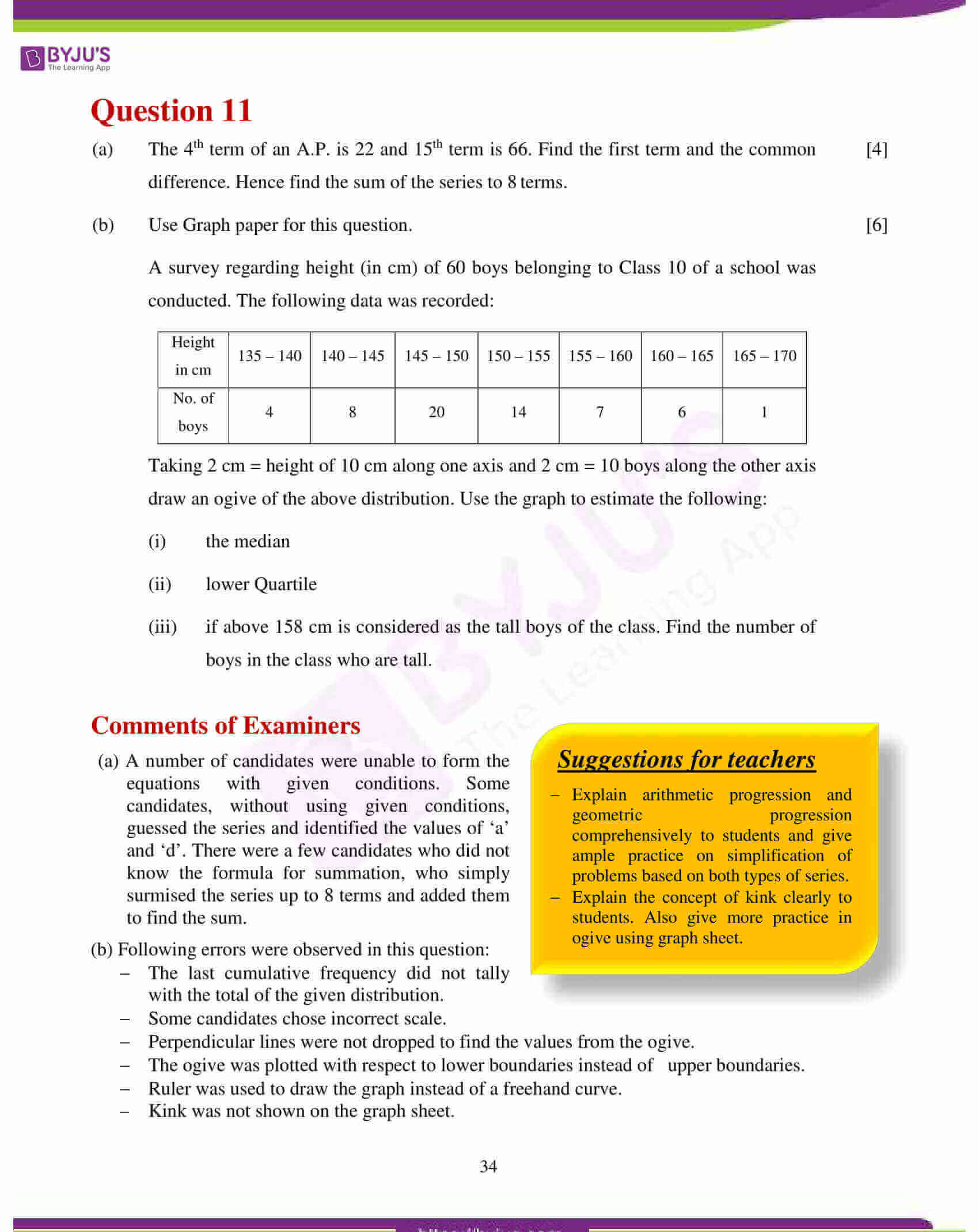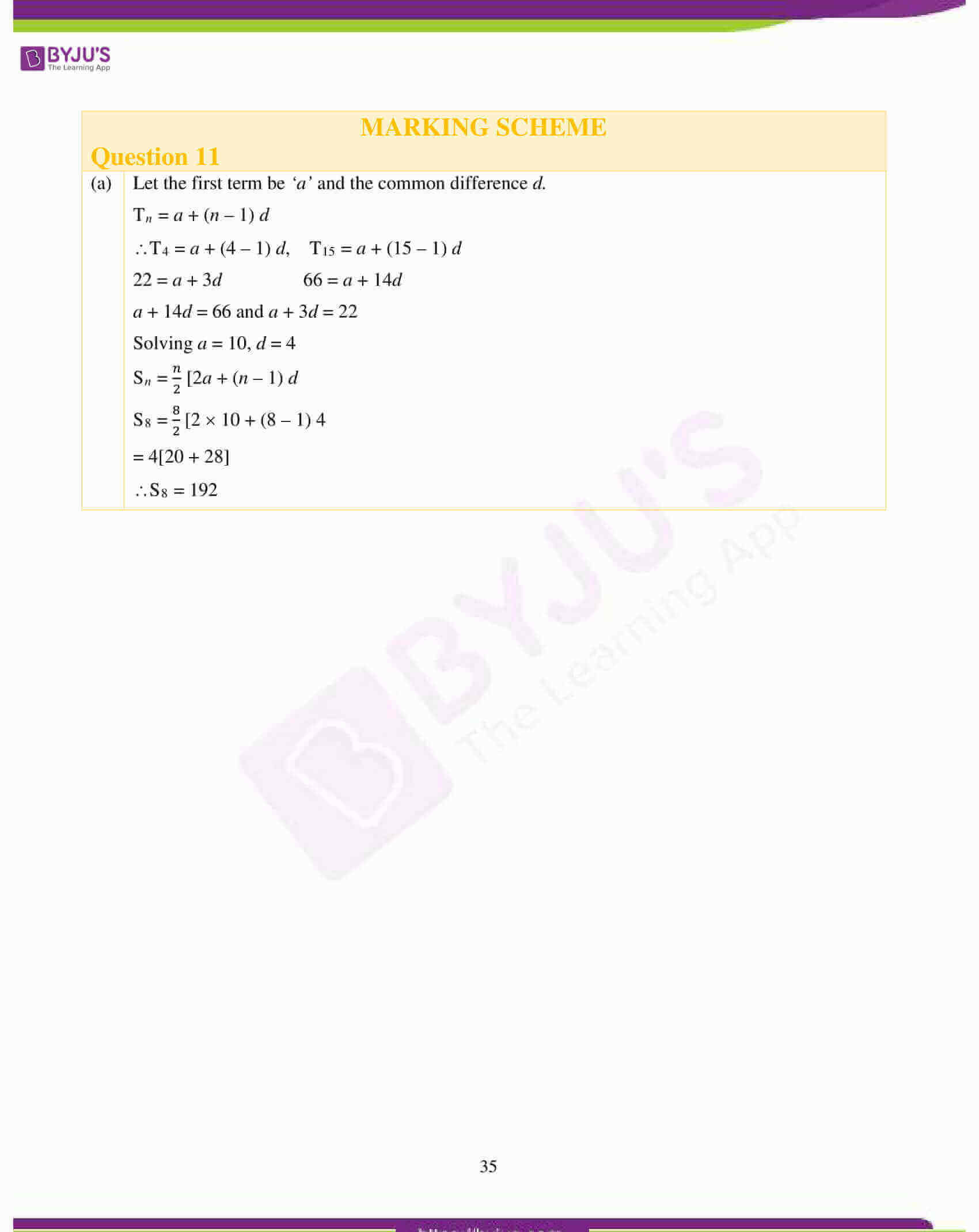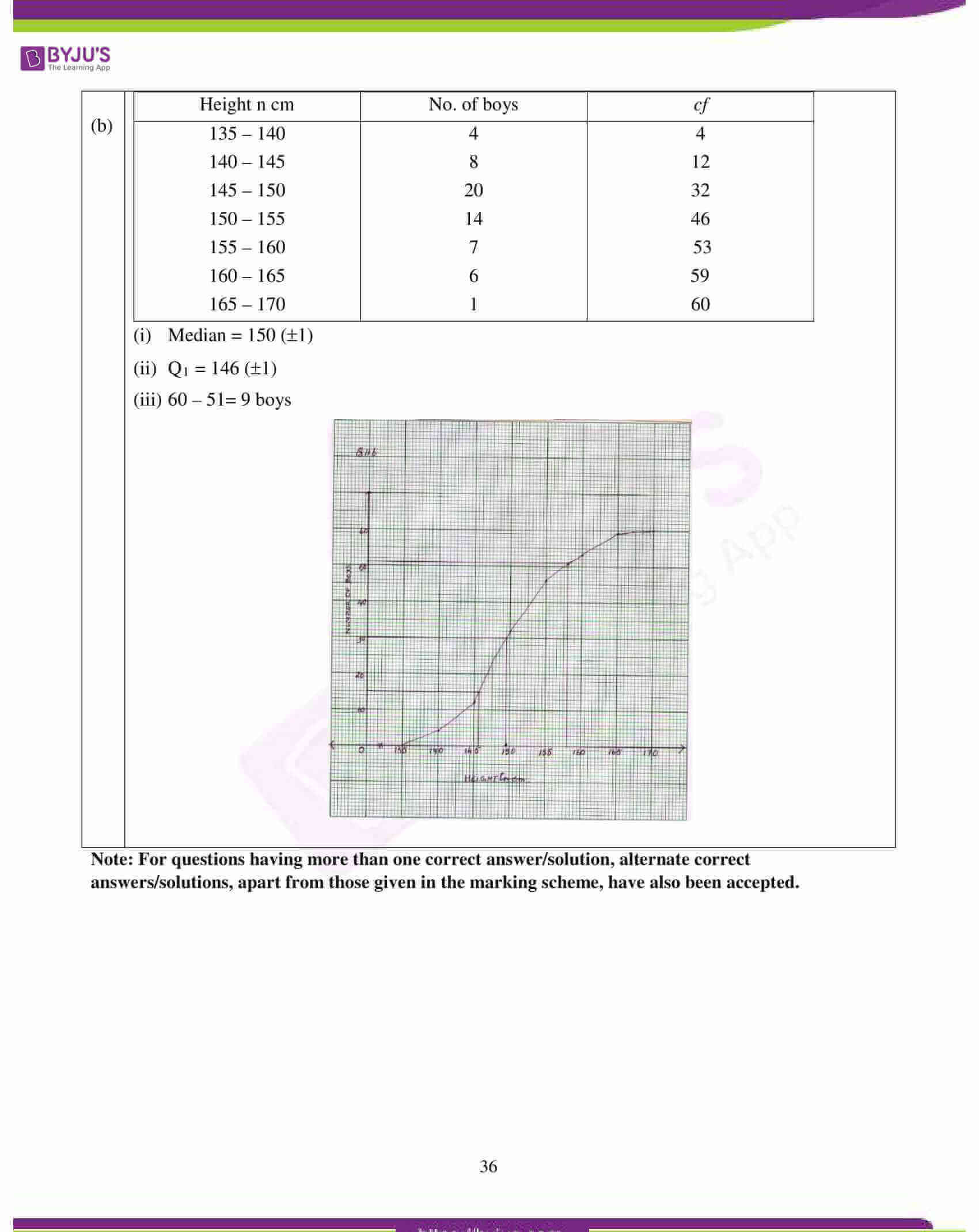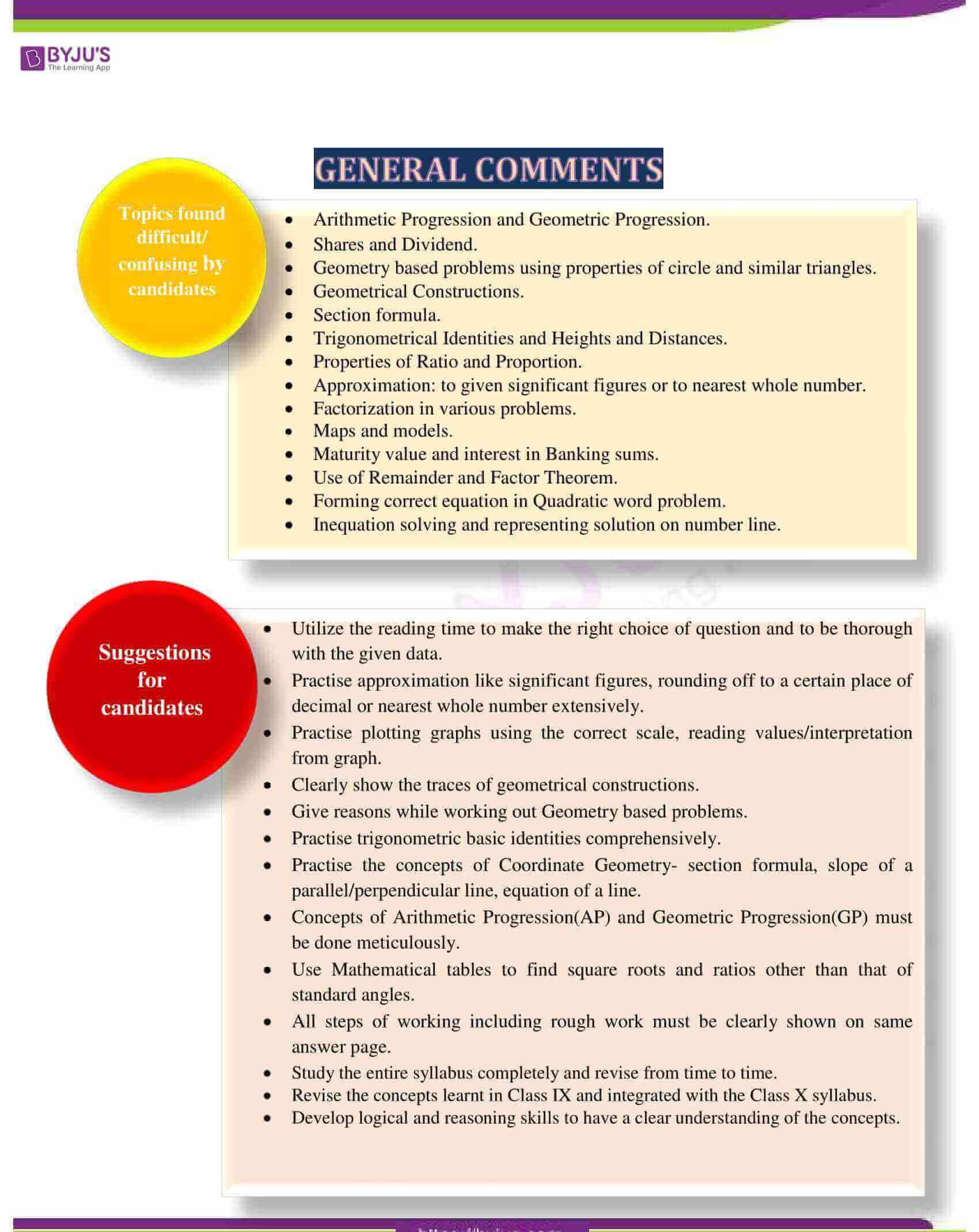## ICSE Class 10 Maths Question Paper 2018 With Solution

### Question 1:

(a) Find the value of ‘x’ and ‘y’ if: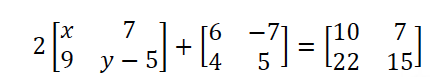(b) Sonia had a recurring deposit account in a bank and deposited ₹600 per month for 2 years and 6 months. If the rate of interest was 10% p.a., find the maturity value of this account.

(c) Cards bearing numbers 2, 4, 6, 8, 10, 12, 14, 16, 18 and 20 are kept in a bag. A card is drawn at random from the bag. Find the probability of getting a card which is:

(i) a prime number.

(ii) a number divisible by 4.

(iii) a number that is a multiple of 6.

(iv) an odd number.

(a)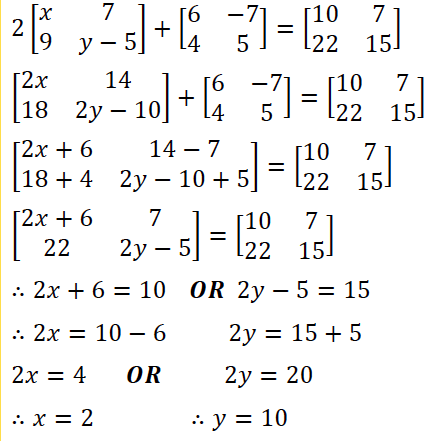(b) Qualifying Sum = 600 × 30 × (30+1) / 2

Interest = 600 x 30 x 31 x 10 / 2 x 12 x 100 = ₹2325

𝑀.𝑉 = 600 x 30 + 2325 = ₹20325

(c) 2, 4, 6, 8, 10, 12, 14, 16, 18, 20

Total number of cards are 10

(i) Probability of getting a prime number is 1/10

(ii) Probability of getting a number divisible by 4:

Numbers are 4, 8, 12, 16 and 20

∴ Probability = 5/10 = ½

(iii) Multiples of 6 are 6, 12, 18.

∴ Probability = 3/10

(iv) No cards bear an odd number.

∴ Probability = 0/10 = 0

### Question 2:

(a) The circumference of the base of a cylindrical vessel is 132 cm and its height is 25 cm. Find the

(ii) volume of cylinder. (use π = 22/7 )

(b) If (k –3), (2k + 1) and (4k + 3) are three consecutive terms of an A.P., find the value of k.

(c) PQRS is a cyclic quadrilateral. Given ∠QPS =73o, ∠PQS = 55o and ∠PSR = 82o, calculate: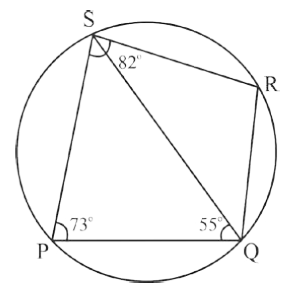(i) ∠QRS

(ii) ∠RQS

(iii) ∠PRQ

(a) 2𝜋𝑟 = 132

𝑟= 21cm

𝑉 = 𝜋 𝑟2ℎ = 22 x 21 x 21 x 25 / 7 = 34650 cm3

(b) (𝑘 − 3), (2𝑘 + 1), (4𝑘 + 3)

𝑘 − 3, 2𝑘 + 1, 4𝑘 + 3 in AP implies

(2𝑘 + 1) − (𝑘 − 3) = (4𝑘 + 3) − (2𝑘 + 1)

∴ 2𝑘 + 1 − 𝑘 + 3 = 4𝑘 + 3 − 2𝑘 − 1

𝑘 + 4 = 2𝑘 + 2

∴ 2𝑘 − 𝑘 = 4 − 2 or 𝑘 = 2

(c)(i) ∠QRS = 180o – 73o = 107o

(ii) ∠RQS = 180 – (82o + 55o)

= 180 – 137

= 43o

(iii) ∠PSQ = 180o – (73o + 55o)

= 52o

∴∠PRQ = ∠PSQ = 52o

### Question 3:

(a) If (𝑥 + 2) and (𝑥 + 3) are factors of 𝑥3 + 𝑎𝑥 + 𝑏, find the values of ‘a’ and ‘b’.

(b) Prove that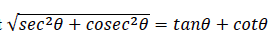(c) Using a graph paper draw a histogram for the given distribution showing the number of runs scored by 50 batsmen. Estimate the mode of the data:

 Runs scored 3000- 4000 4000- 5000 5000- 6000 6000- 7000 7000- 8000 8000- 9000 9000- 10000 No. of batsmen 4 18 9 6 7 2 4

(a)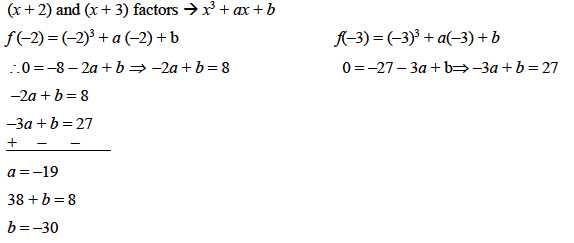(b)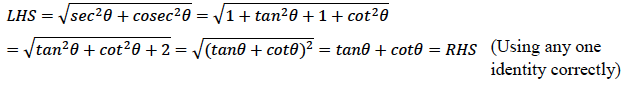(c) Mode = 4600 runs

Axis and Scale: On X axis: 2 cm = 1000 runs

On Y axis: 2 cm = 4 batsmen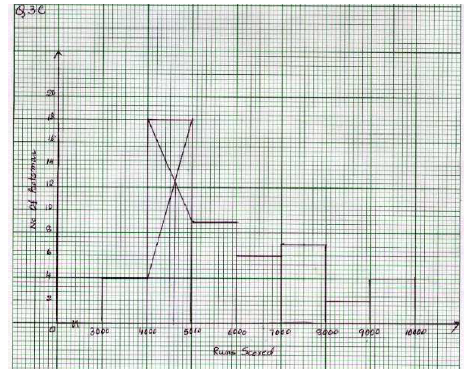### Question 4:

(a) Solve the following inequation, write down the solution set and represent it on the

real number line: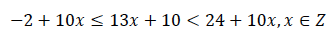(b) If the straight lines 3𝑥 − 5𝑦 = 7 and 4𝑥 + 𝑎y + 9 = 0 are perpendicular to one another, find the value of a.

(c) Solve 𝑥2 + 7𝑥 = 7 and give your answer correct to two decimal places.

(a)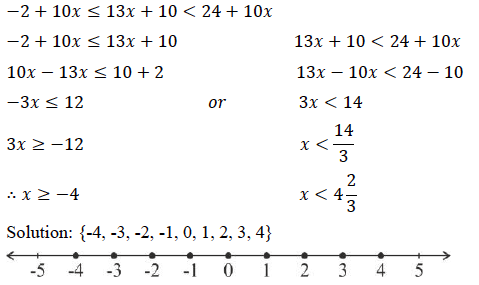(b)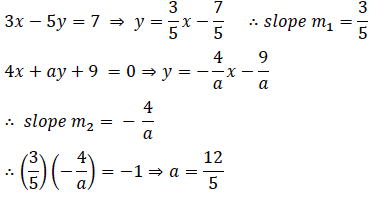(c)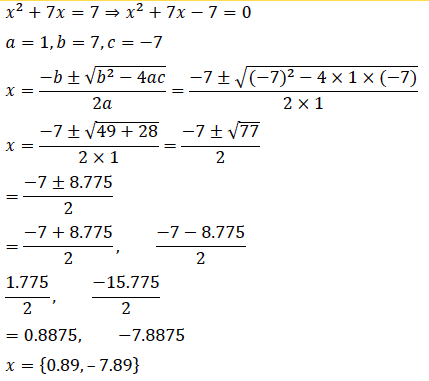### Question 5:

(a) The 4th term of a G.P. is 16 and the 7th term is 128. Find the first term and common ratio of the series.

(b) A man invests ₹22,500 in ₹50 shares available at 10% discount. If the dividend paid by the company is 12%, calculate:

(i) The number of shares purchased

(iii) The rate of return he gets on his investment. Give your answer correct to the nearest whole number.

(c) Use graph paper for this question (Take 2 cm = 1unit along both x and y axis). ABCD is a quadrilateral whose vertices are A (2,2), B (2, –2), C (0,–1) and D(0,1).

(i) Reflect quadrilateral ABCD on the y-axis and name it as A’B’CD.

(ii) Write down the coordinates of A’ and B’.

(iii) Name two points which are invariant under the above reflection.

(iv) Name the polygon A’B’CD.

(a)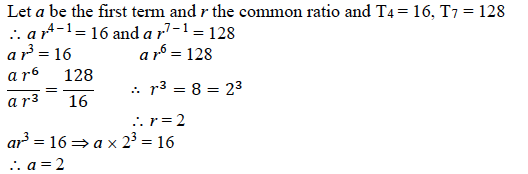(b) NV = 50

MV= 50 – 10% of 50 = 45

(i) Number of shares = 22500/45 = 500

(ii) Annual dividend = 12x50x500/100 = 3000

(iii) Yield% = 3000/22500 x 100 = 13.333% ≈ 13%

(c) (i) Quadrilateral A’B’CD marked on graph sheet

(ii) A'(–2, 2) and B'(–2, –2)

(iii) C and D

(iv) Isosceles trapezium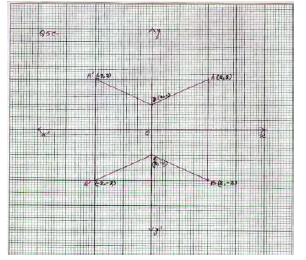### Question 6:

(a) Using properties of proportion, solve for x. Given that x is positive: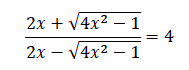(b)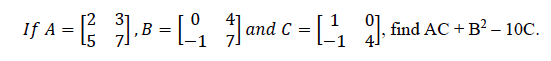(c) Prove that (1 + cot 𝜃 − cosec 𝜃)(1 + tan 𝜃 + sec 𝜃) = 2

(a)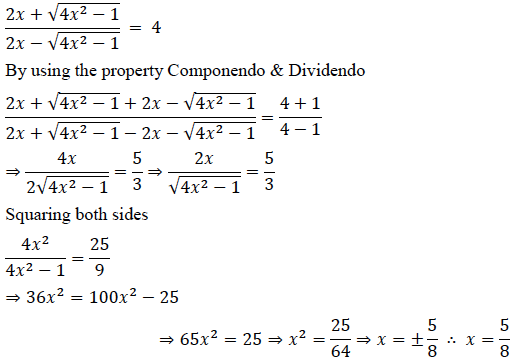(b)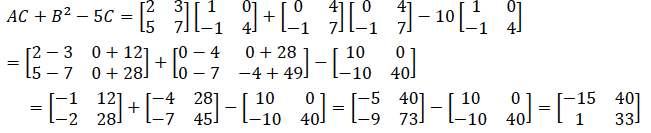(c)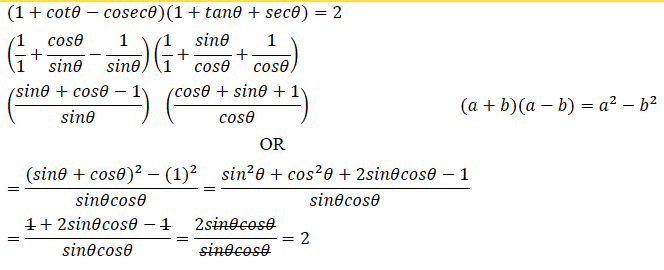### Question 7:

(a) Find the value of k for which the following equation has equal roots.

𝑥2 + 4𝑘 + (𝑘2 − 𝑘 + 2) = 0

(b) On a map drawn to a scale of 1:50,000, a rectangular plot of land ABCD has the following dimensions. AB = 6 cm; BC = 8 cm and all angles are right angles.

Find:

(i) the actual length of the diagonal distance AC of the plot in km.

(ii) the actual area of the plot in square km.

(c) A (2, 5), B (–1, 2) and C (5, 8) are the vertices of a triangle ABC, ‘M’ is a point on AB such that AM: MB = 1: 2. Find the co-ordinates of ‘M’. Hence find the equation of the line passing through the points C and M.

(a) Given equation is x2 + 4kx + (k2 – k + 2) = 0

For equal roots discriminant = 0

∴ (4k)2 – 4(k2 – k + 2) = 0

16k2 – 4k2 + 4k – 8 = 0

12k2 + 4k – 8 = 0

3k2 + k – 2 = 0 ⇒ 3k2 + 3k – 2k = 0

3k(k + 1)(3k – 2) = 0

∴ k = –1, k = 2/3

(b)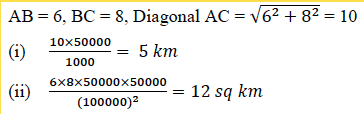(c)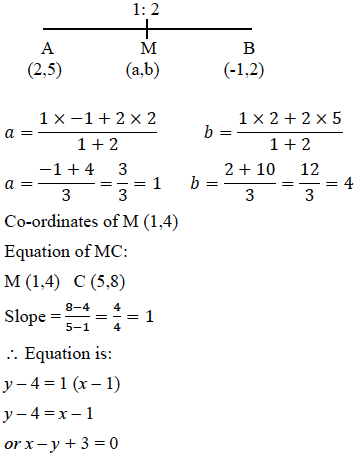### Question 8:

(a) ₹7500 were divided equally among a certain number of children. Had there been 20 less children, each would have received ₹100 more. Find the original number of children.

(b) If the mean of the following distribution is 24, find the value of ‘a’.

 Marks 0 – 10 10 – 20 20 – 30 30 – 40 40 – 50 Number of students 7 a 8 10 5

(c) Using ruler and compass only, construct a ΔABC such that BC = 5 cm and AB = 6.5 cm and ∠ABC = 1200

(i) Construct a circum-circle of ΔABC

(ii) Construct a cyclic quadrilateral ABCD, such that D is equidistant from AB and BC.

(a) Let the original number of children be x.

∴ each one gets 7500/𝑥

When 20 children less, each would get 7500/(𝑥−20)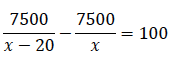⇒ 𝑥2 − 20𝑥 − 1500 = 0

⇒ (𝑥 − 50)(𝑥 + 30) = 0

∴ 𝑥 = 50

(b)

 Marks No. of Students (f) x fx 0 – 10 7 5 35 10 – 20 a 15 15a 20 – 30 8 25 200 30 – 40 10 35 350 40 – 50 5 45 225 Σf = 30 + a 810 + 15a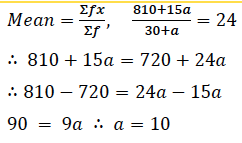(c) Construction of ΔABC

Construction of side bisectors of sides AB and BC and draw circle

Angle bisector of angle B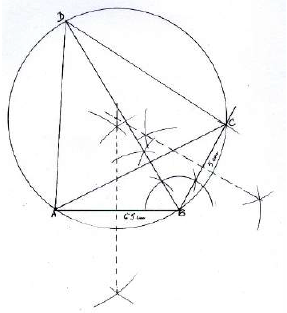### Question 9:

(a) Priyanka has a recurring deposit account of ₹1000 per month at 10% per annum. If she gets ₹5550 as interest at the time of maturity, find the total time for which the account was held.

(b) In ΔPQR, MN is parrallel to QR and

𝑃𝑀/MQ = 2/3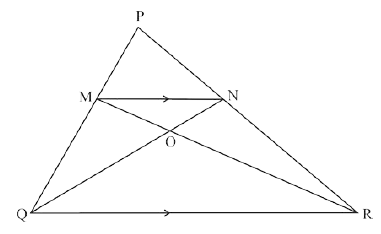(i) Find MN/QR

(ii) Prove that ΔOMN and ΔORQ are similar.

(iii) Find, Area of ΔOMN : Area of ΔORQ

(c) The following figure represents a solid consisting of a right circular cylinder with a hemisphere at one end and a cone at the other. Their common radius is 7 cm. The height of the cylinder and cone are each of 4 cm. Find the volume of the solid.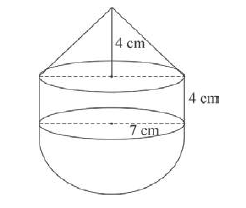(a) Monthly Deposit = ₹1000, rate of interest 10%

Total interest = ₹5550

Let n be the number of months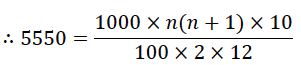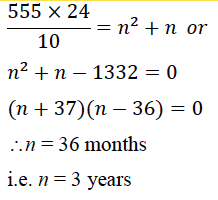(b) Proving ΔPMN ~ ΔPQR(i) MN/QR = 2/5

(ii) ∠MON = ∠QOR (vertically opposite angles)

∠MNO = ∠OQR (alternate angles)

∠NMO = ∠ORQ (3rd angle)

(iii) ar ΔOMN: ar ΔORQ = 4 : 25

(c)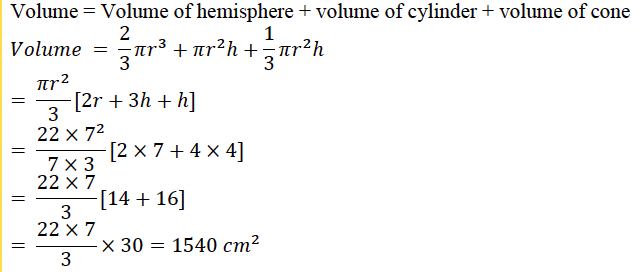### Question 10:

(a) Use Remainder theorem to factorize the following polynomial:

2𝑥3 + 3𝑥2 − 9𝑥 − 10.

(b) In the figure given below ‘O’ is the centre of the circle. If QR = OP and ∠ORP = 200. Find the value of ‘x’ giving reasons.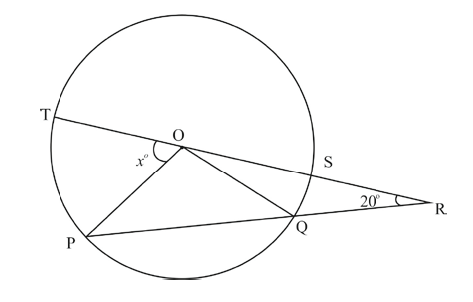(c) The angle of elevation from a point P of the top of a tower QR, 50 m high is 60o and that of the tower PT from a point Q is 30o. Find the height of the tower PT, correct to the nearest metre.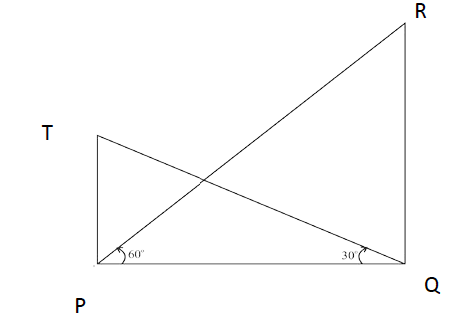(a)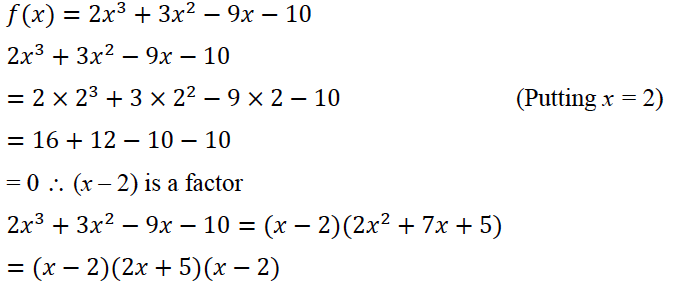(b) ∵ OP = OQ (radii of circle)

And OP = QR (given )

∴ OP = OQ = QR

In ΔOQR, OQ = QR

∴ ∠ORQ = ∠ROQ = 200

ext. ∠OQP = 200 + 200 = 400 (exterior angle = sum of 2 interior opposite angles)

In ΔOPQ, OP = OQ (radii)

∴ ∠OPQ = ∠OQP = 400

In ΔOPQ, ext ∠POT = ∠OPR + ∠ORP = 400+ 200 = 600

(c) In ΔPQR,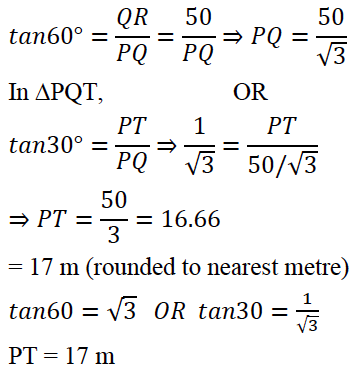### Question 11:

(a) The 4th term of an A.P. is 22 and 15th term is 66. Find the first term and the common difference. Hence find the sum of the series to 8 terms.

(b) Use Graph paper for this question.

A survey regarding height (in cm) of 60 boys belonging to Class 10 of a school was

conducted. The following data was recorded:

 Height in cm 135 –140 140 –145 145 –150 150 –155 155 –160 160 –165 165 –170 No. of boys 4 8 20 14 7 6 1

Taking 2 cm = height of 10 cm along one axis and 2 cm = 10 boys along the other axis draw an ogive of the above distribution. Use the graph to estimate the following:

(i) the median

(ii) lower Quartile

(iii) if above 158 cm is considered as the tall boys of the class. Find the number of boys in the class who are tall.

(a)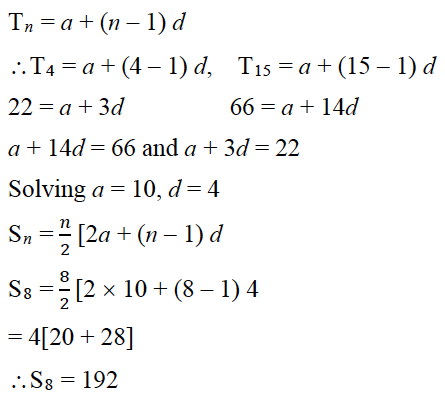(b)

 Height n cm No. of boys cf 135 – 140 4 4 140 – 145 8 12 145 – 150 20 32 150 – 155 14 46 155 – 160 7 53 160 – 165 6 59 165 – 170 1 60

(i) Median = 150 (±1)

(ii) Q1 = 146 (±1)

(iii) 60 – 51= 9 boys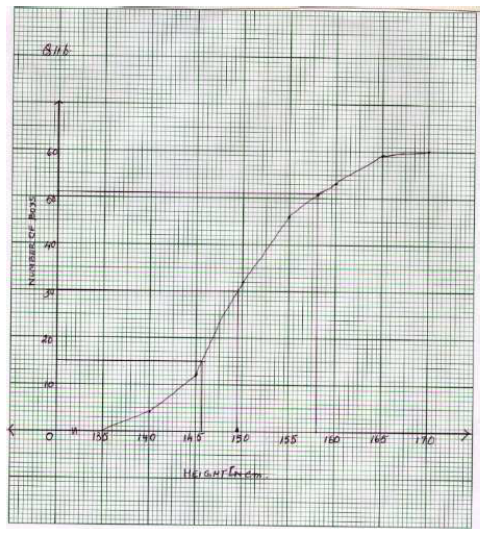We hope students found this information on “ICSE Class 10 Maths Question Papers Solutions 2018” helpful for their exam preparation. They can also access solutions of other subjects of ICSE Class 10 Previous Years Question Papers by clicking here. Stay tuned to BYJU’S for the latest update on ICSE/CBSE/State Boards/Competitive exams. Also, download the BYJU’S App and fall in love with learning.